## Friday, January 04, 2019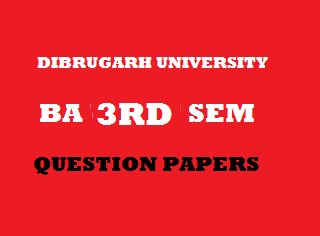2014
(November)
MATHEMATICS
(Major)
Course: 301
[Analysis – I (Real Analysis)]
Full Marks: 80
Pass Marks: 32
Time: 3 hours
The figures in the margin indicate full marks for the questions
GROUP – A
(Differential Calculus)
(Marks: 35)

1. (a) If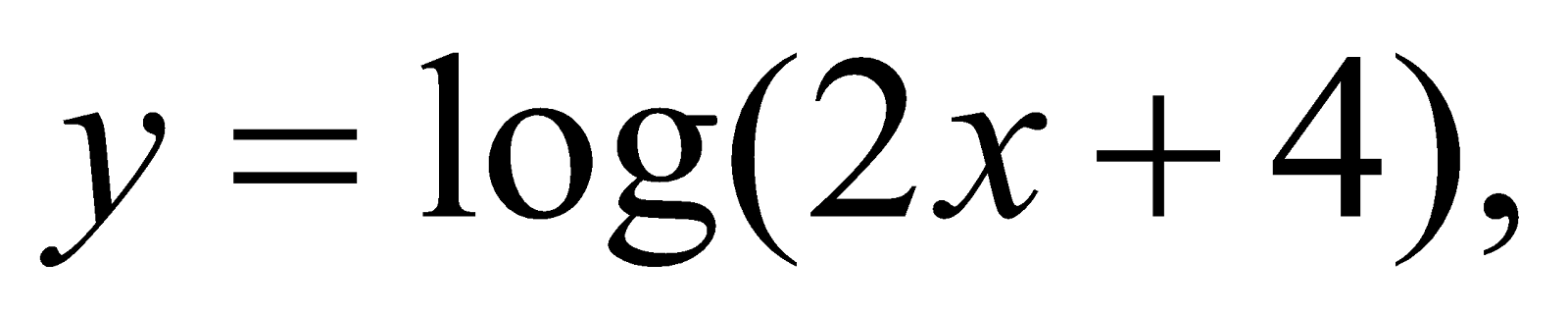then write the value of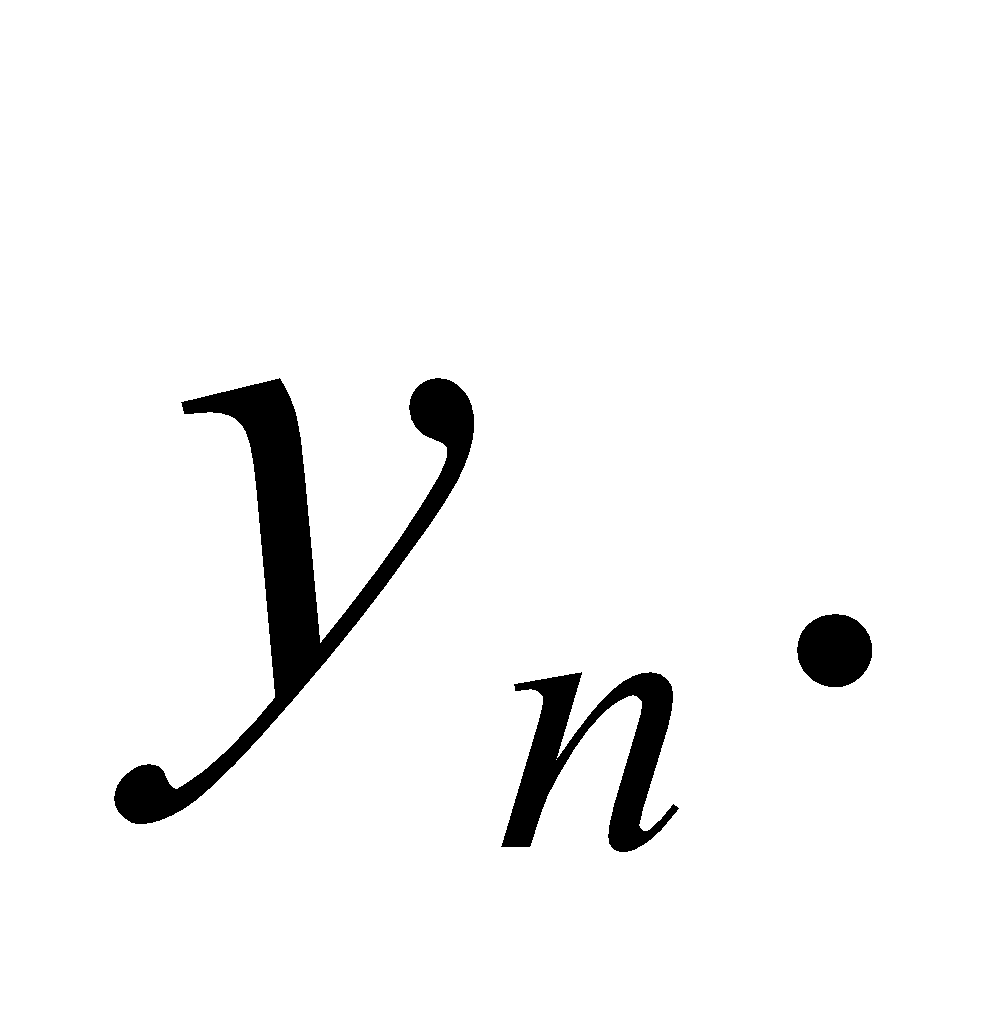1
(b) Find the limit: 2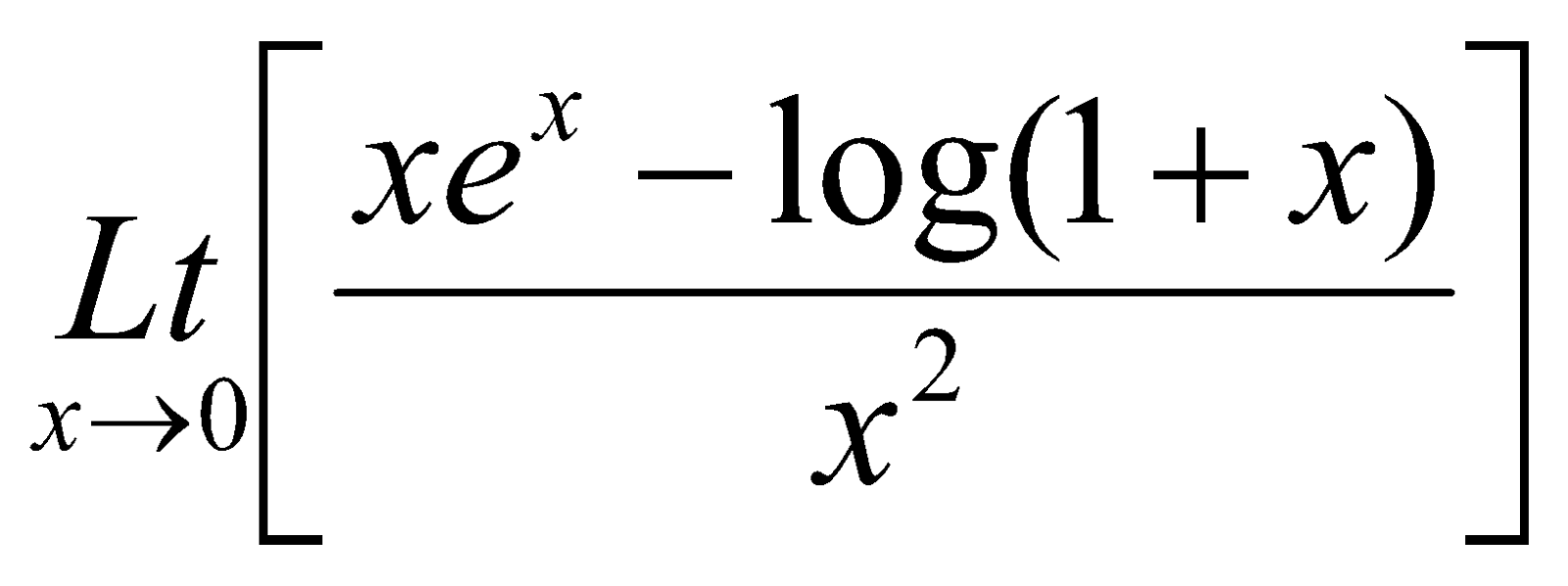(c) Define sub tangent. Show that the sub tangent at any point of a parabola varies as the abscissa of the point of contact. 1+2=3
(d) If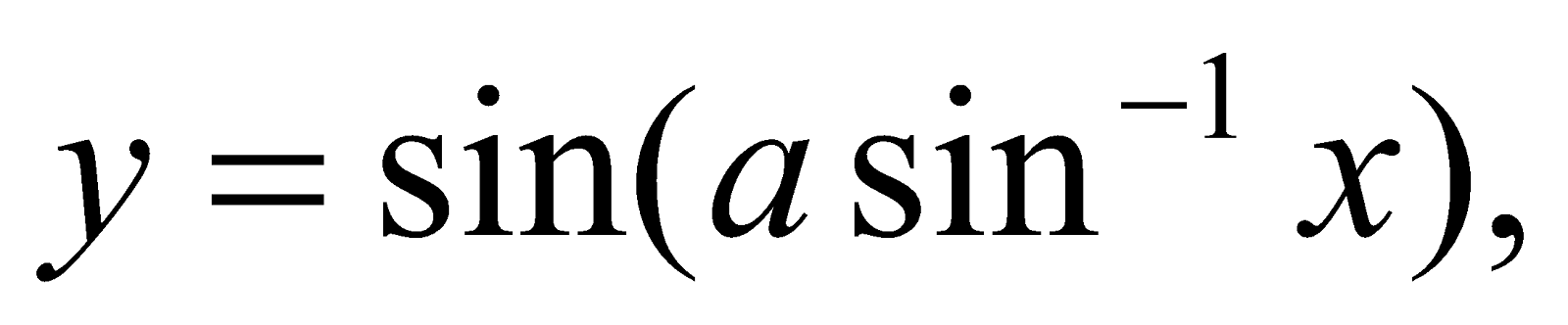then prove that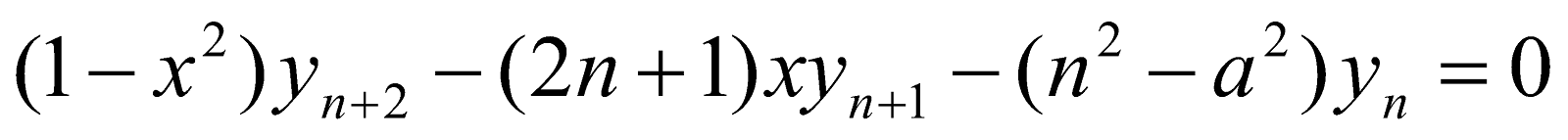Or
Show that the radius of curvature at the origin of the conic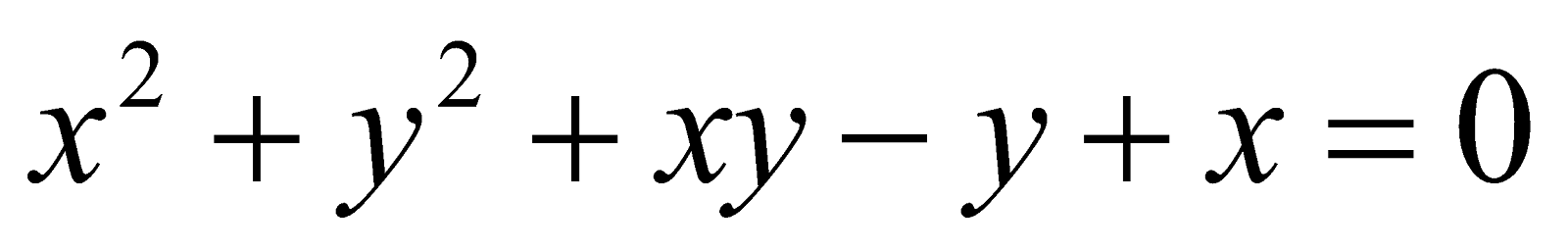is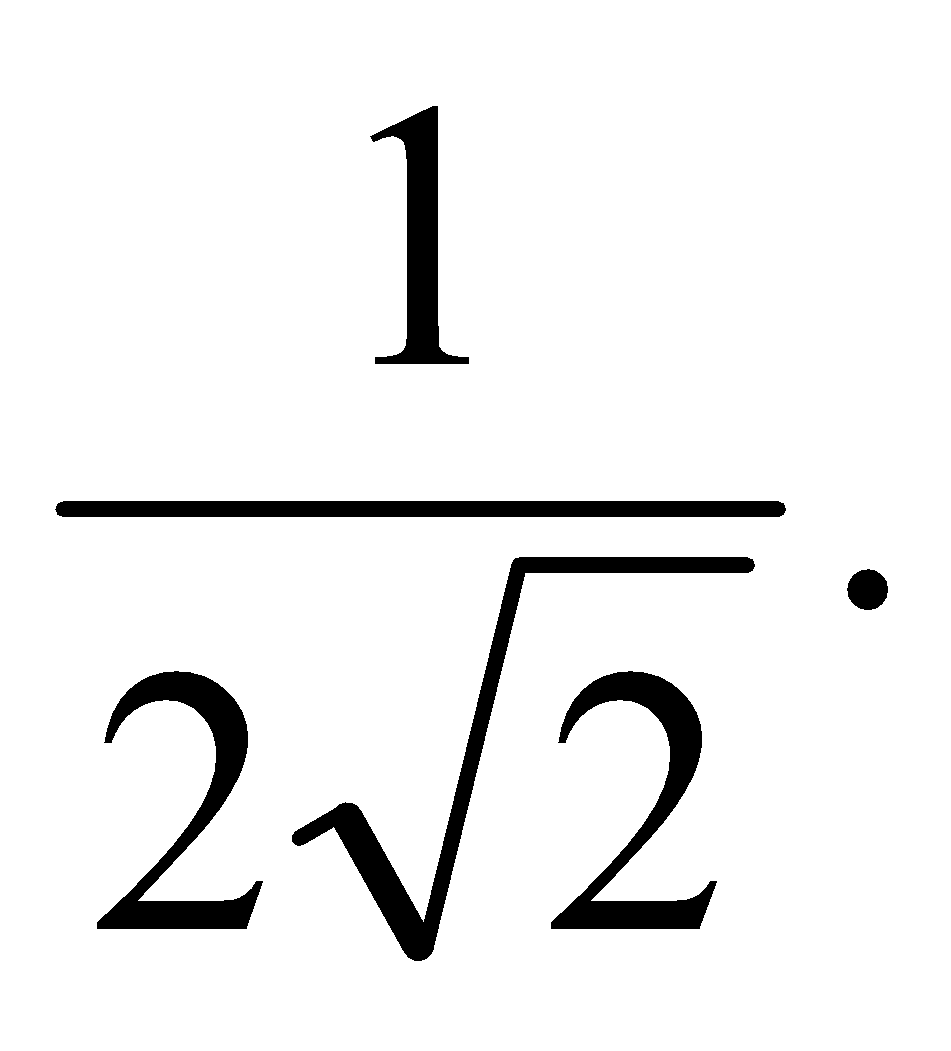2. (a) Give an example of a continous function in a domain which has neither infimum nor supremum therein.
(b) If a function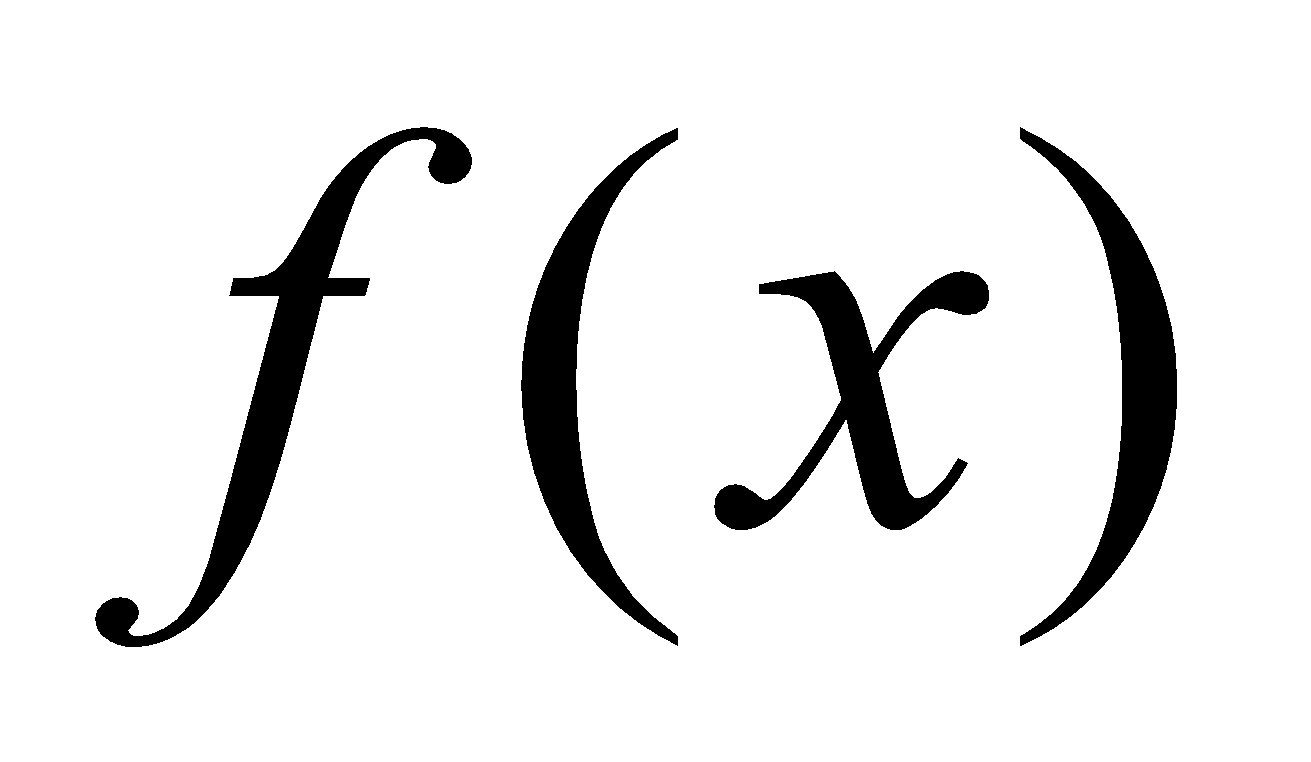satisfies the conditions of Lagrange’s mean value theorem and also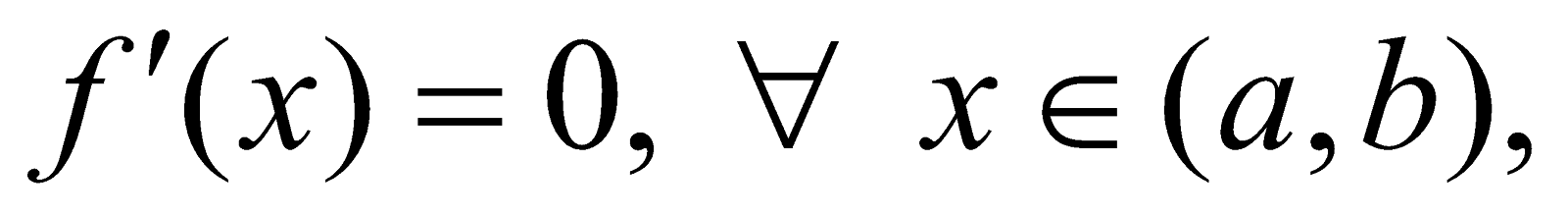then show thatis constant on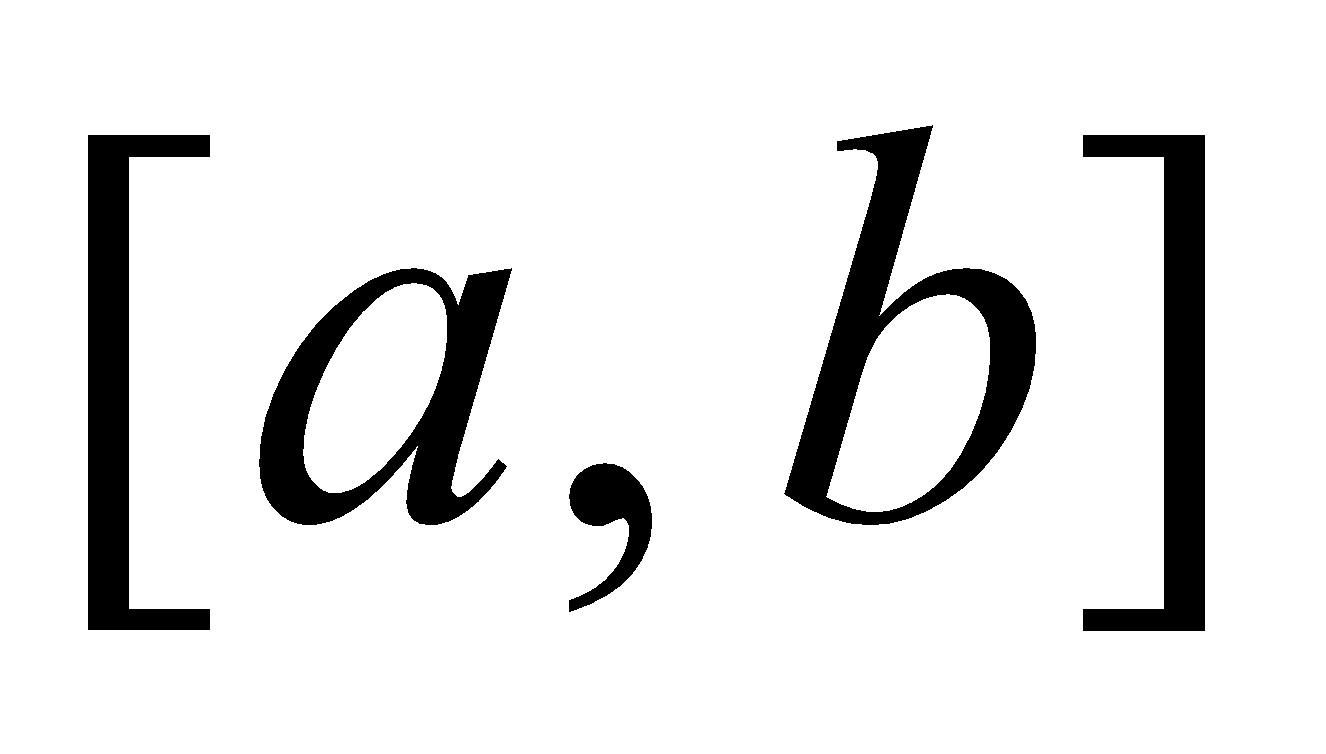.
(c) If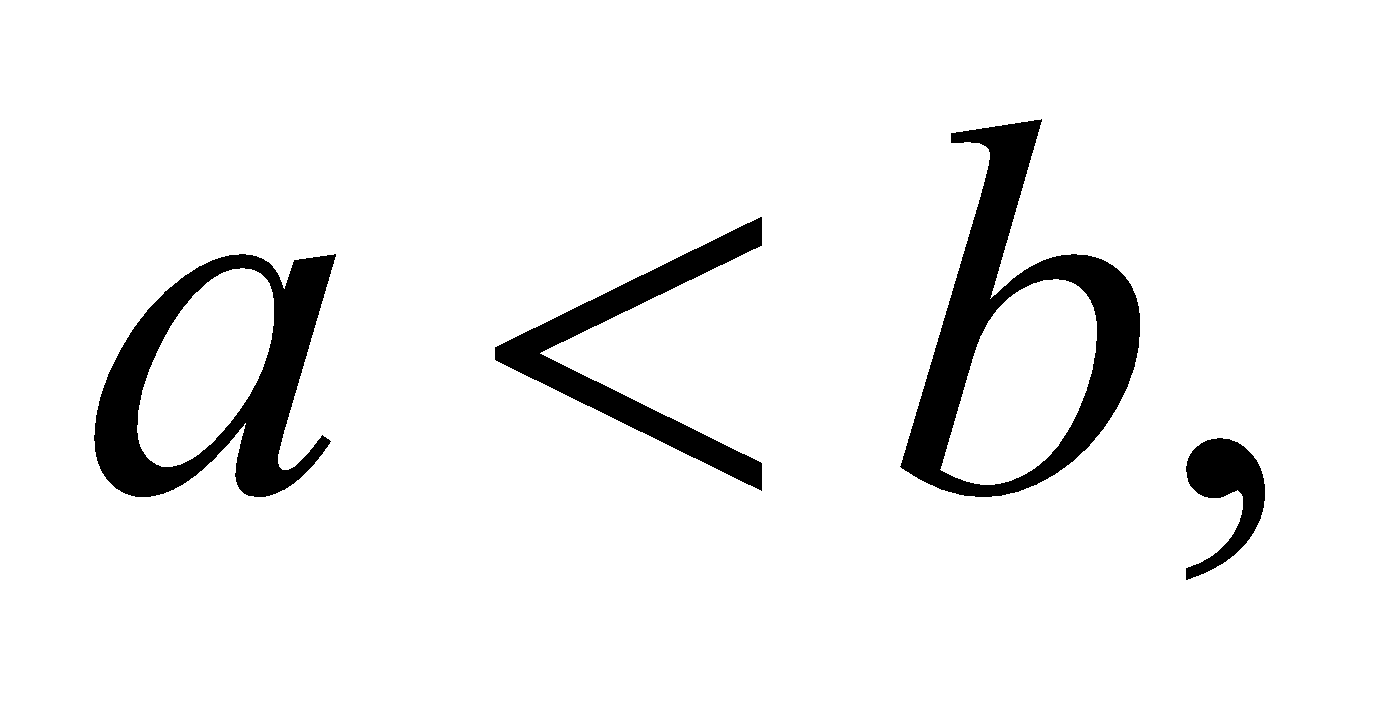then prove that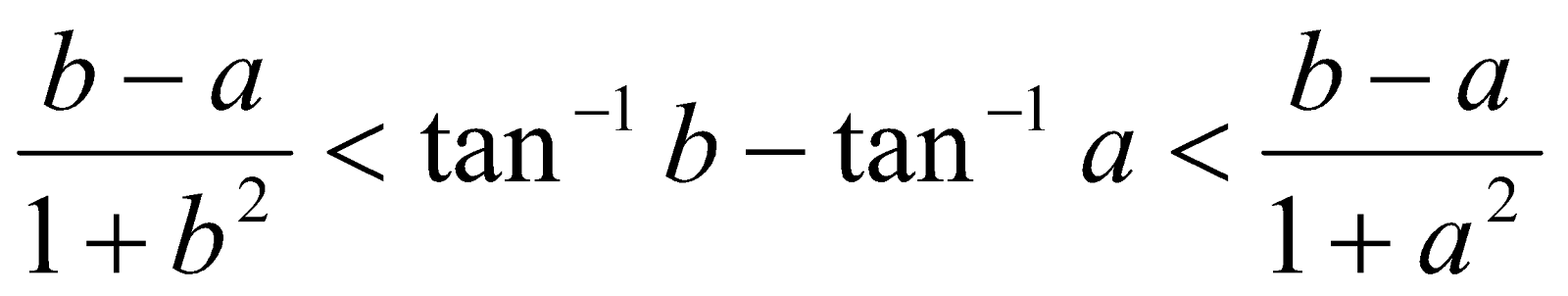Or
Using Maclaurin’s theorem, expand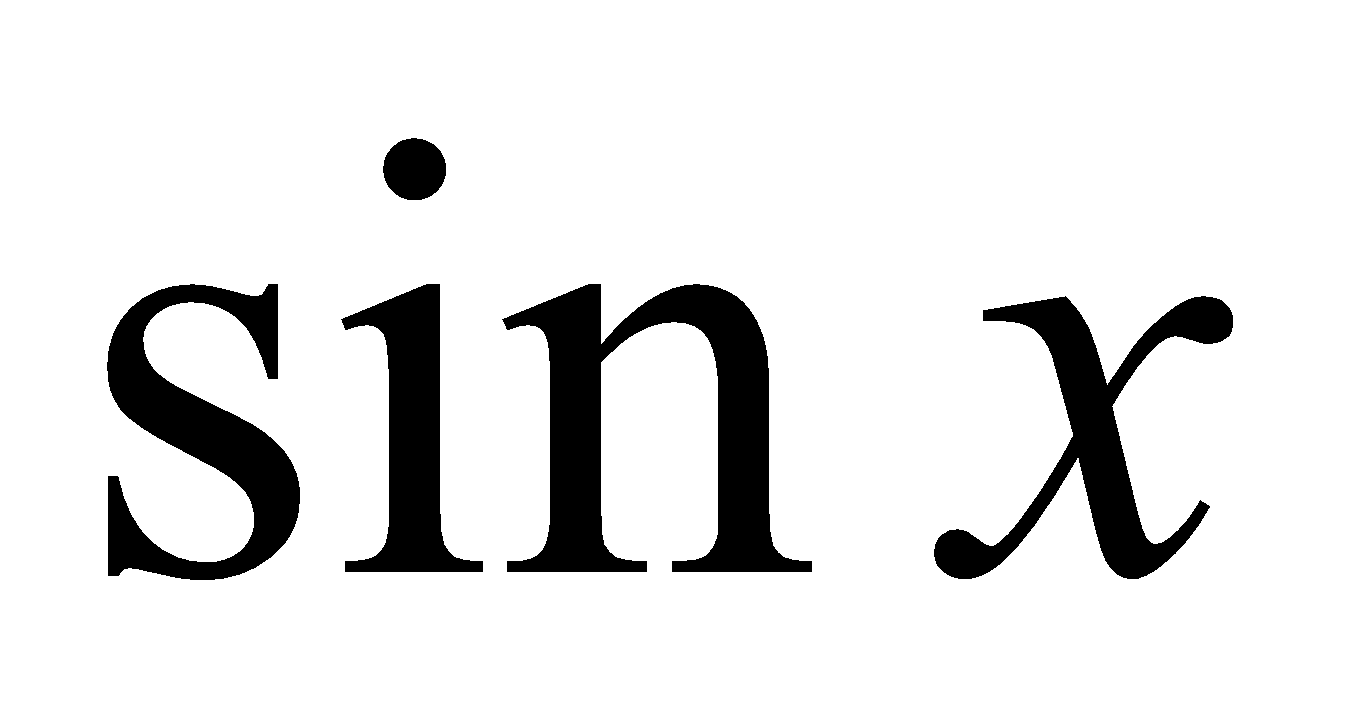in an infinite series.
(d) Discuss the applicability of the Rolle’s Theorem for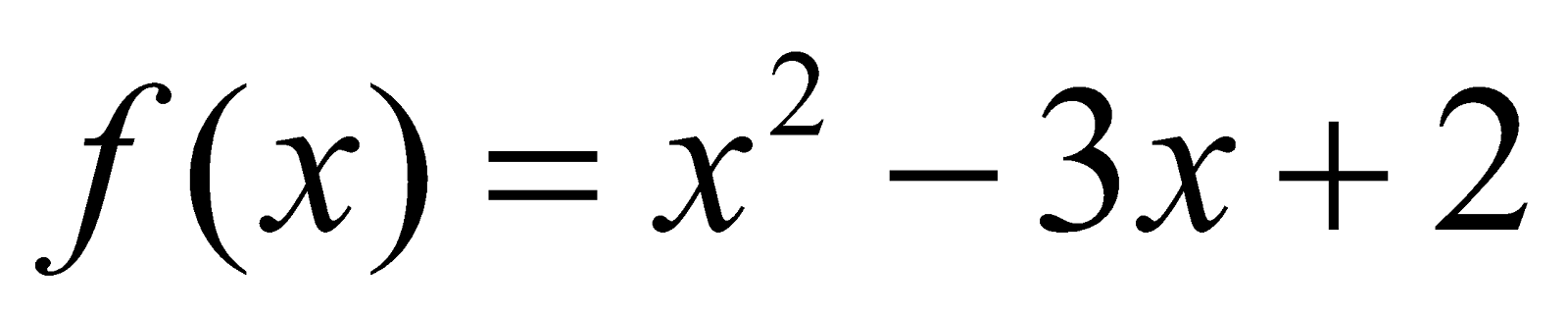in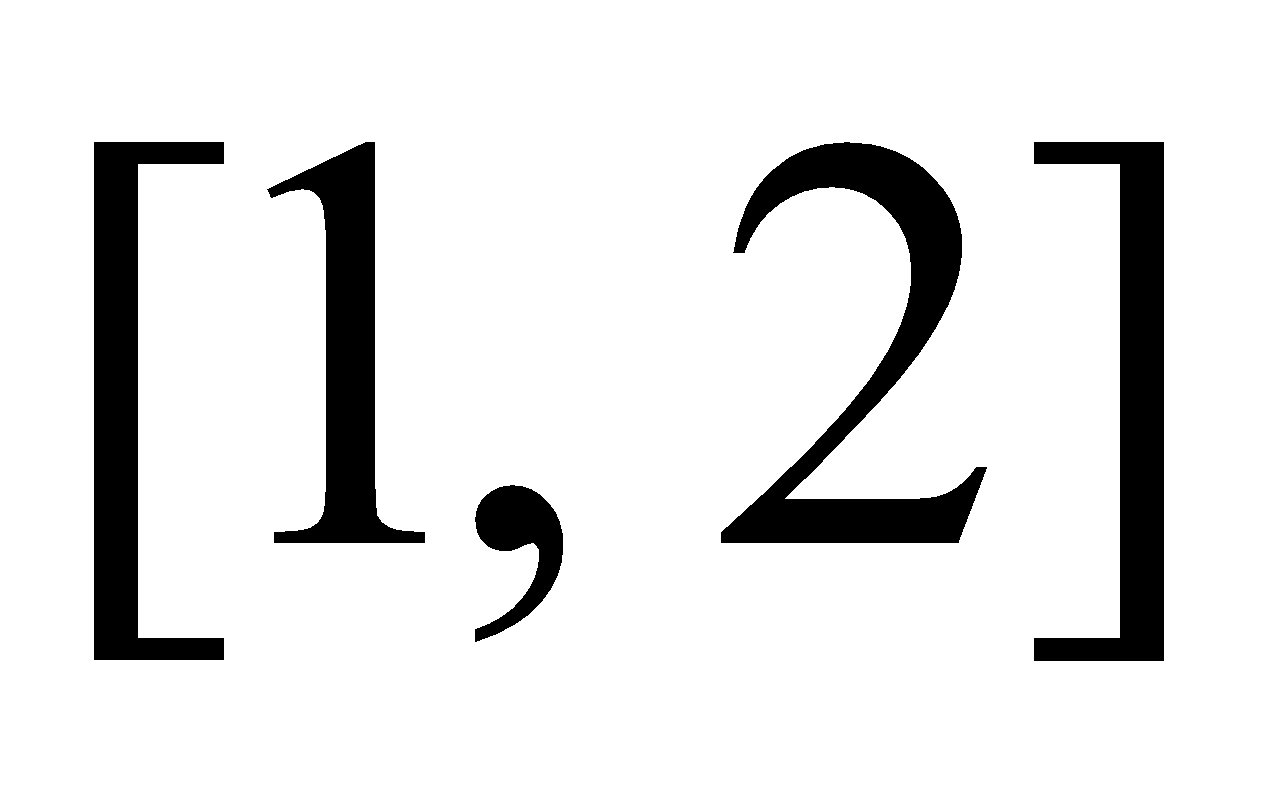.
3. (a) Define homogeneous function of two variables. 1
(b) If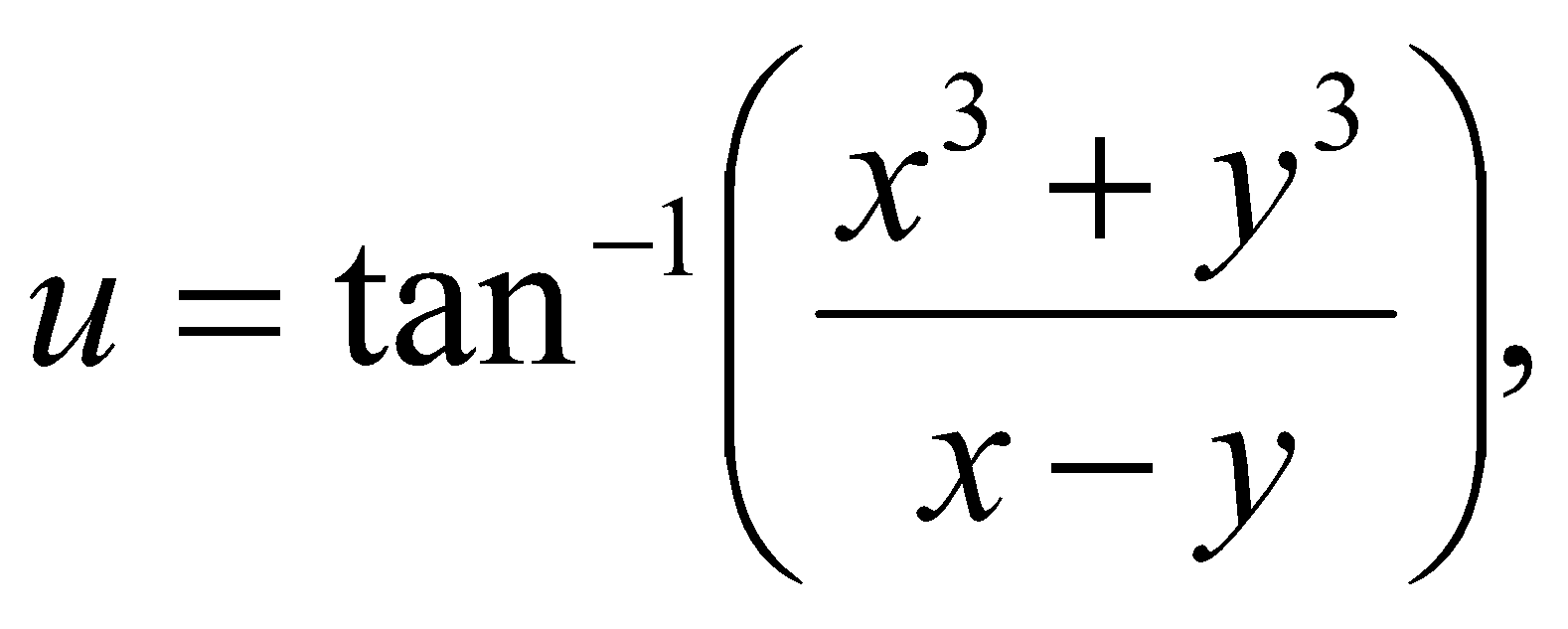then show that 4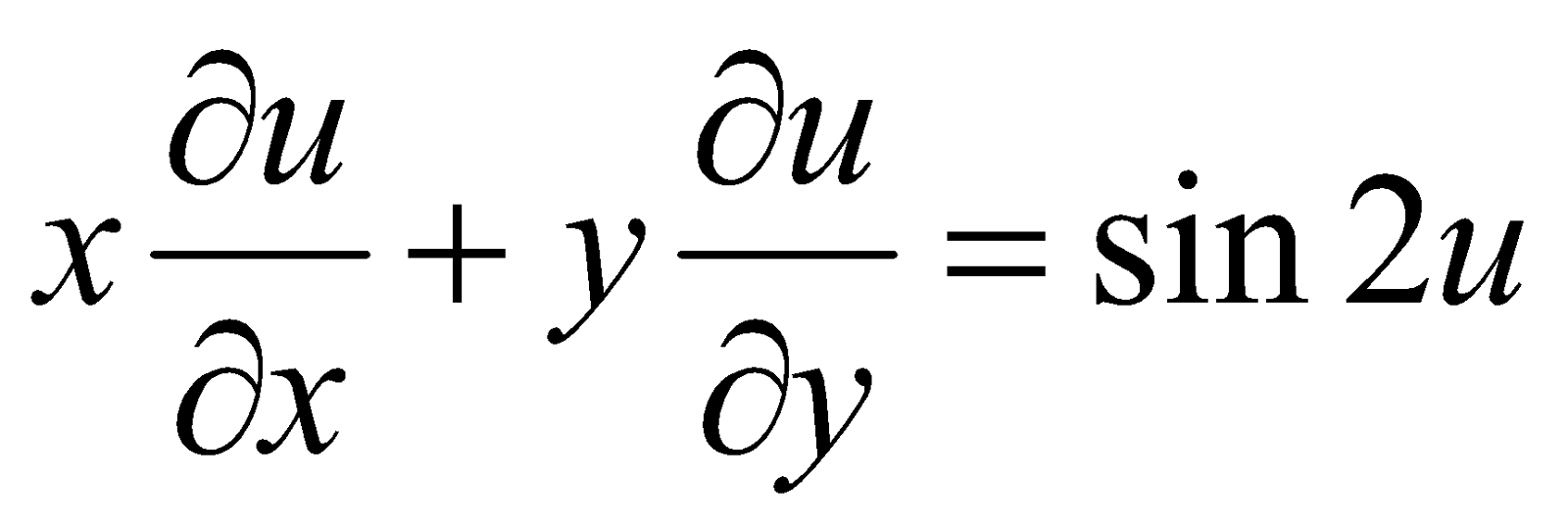Or
If u is a homogeneous function, of degree n, of x and y, then show that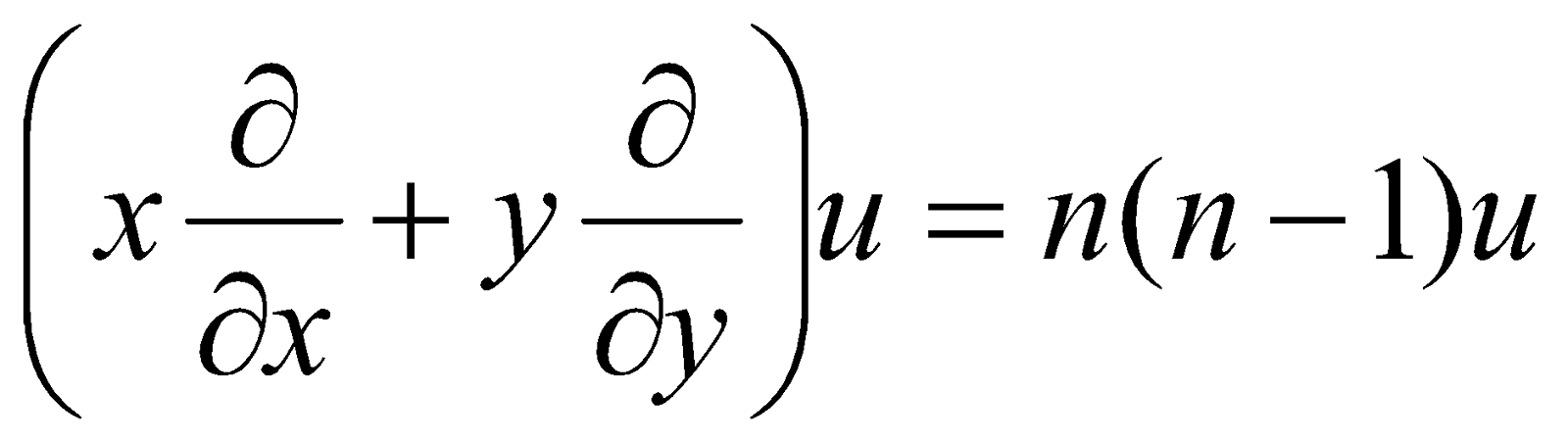4. (a) State Young’s theorem. 1
(b) If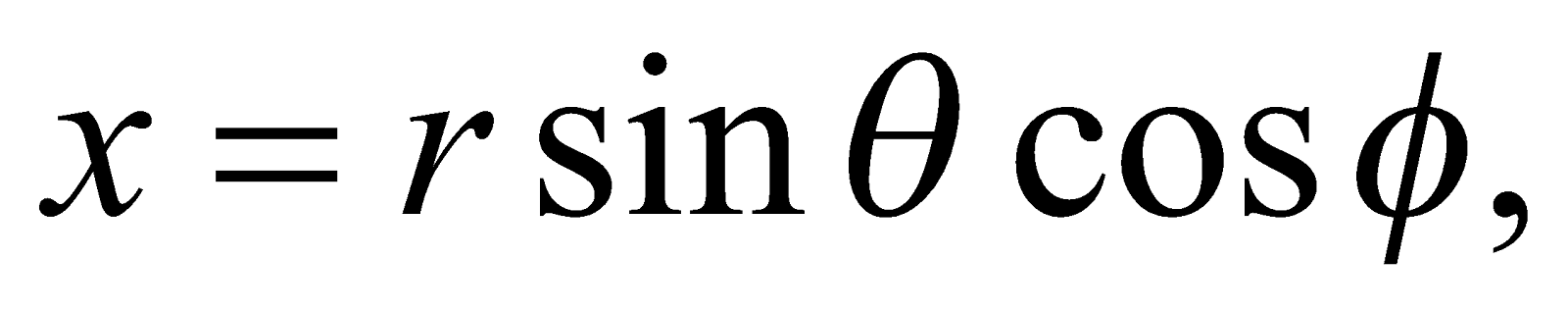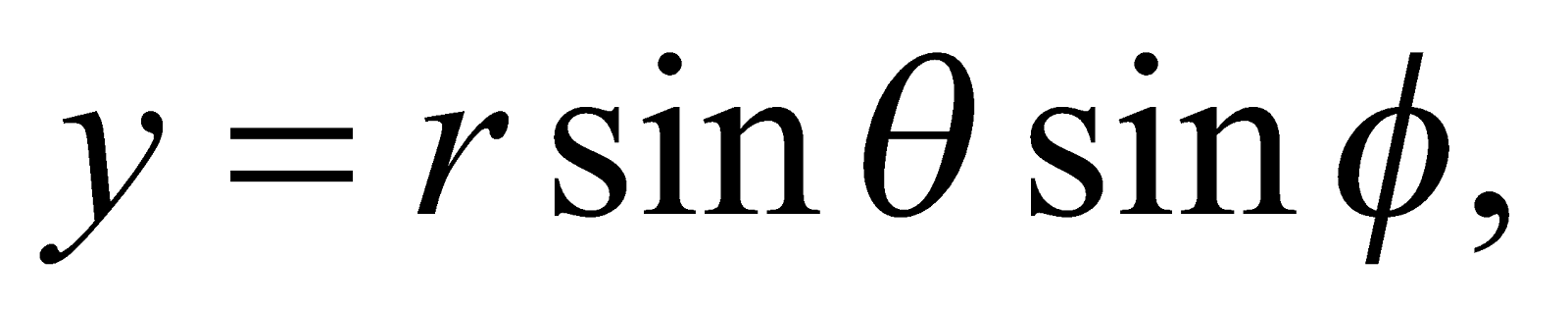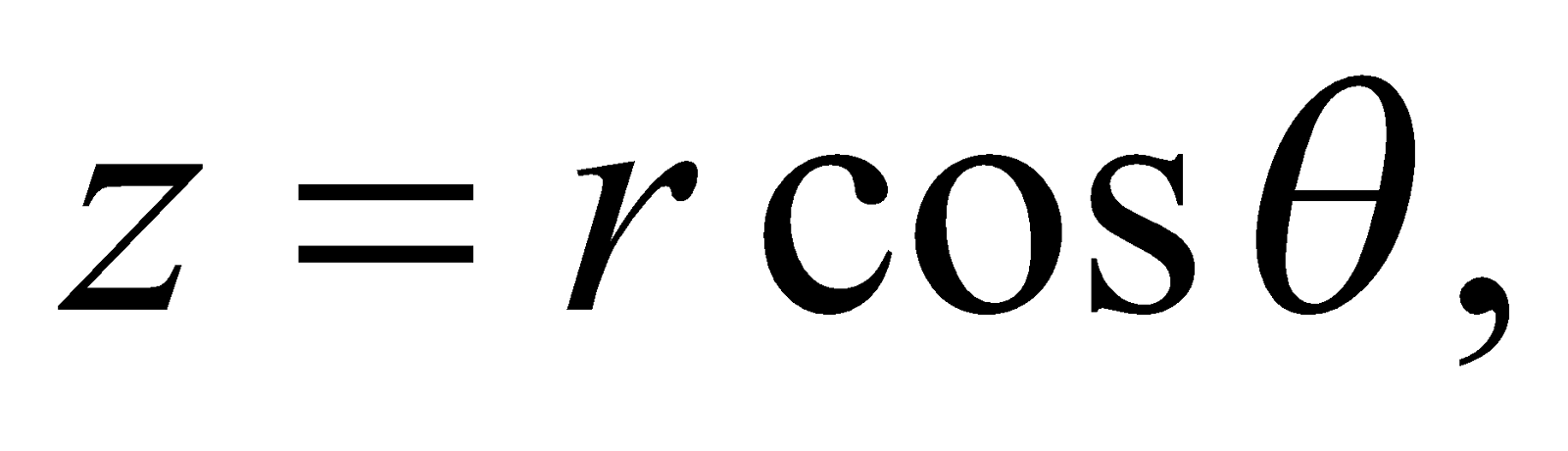then show that 4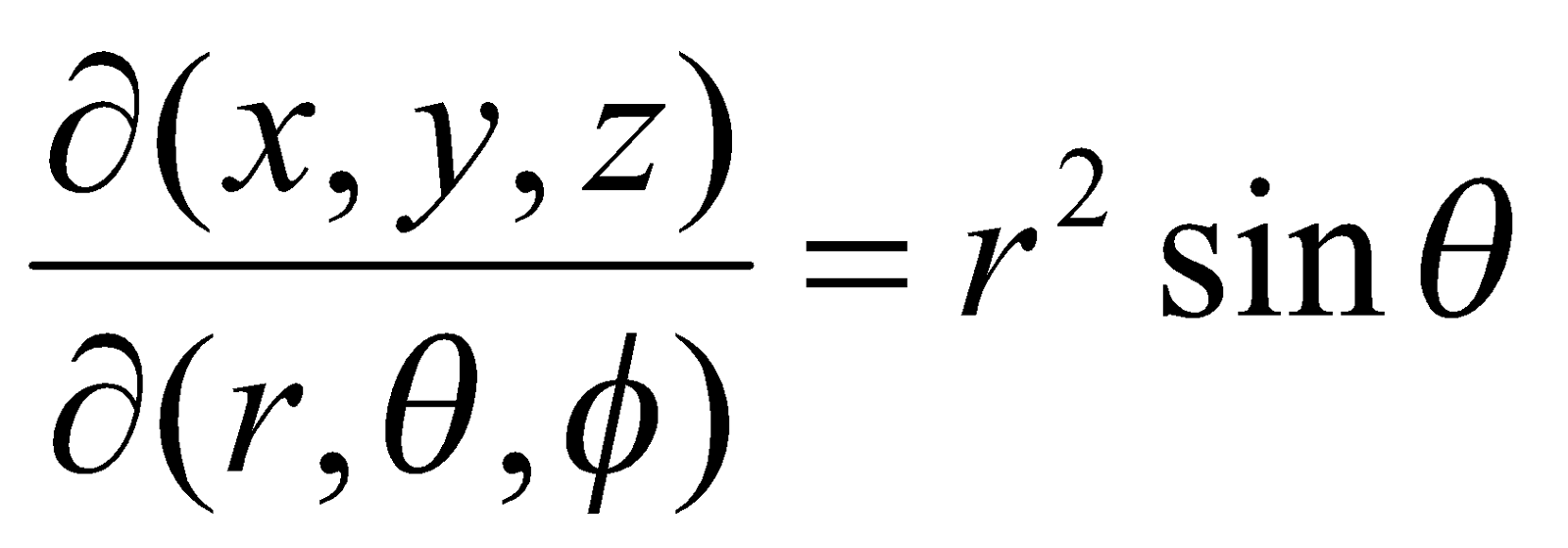(c) If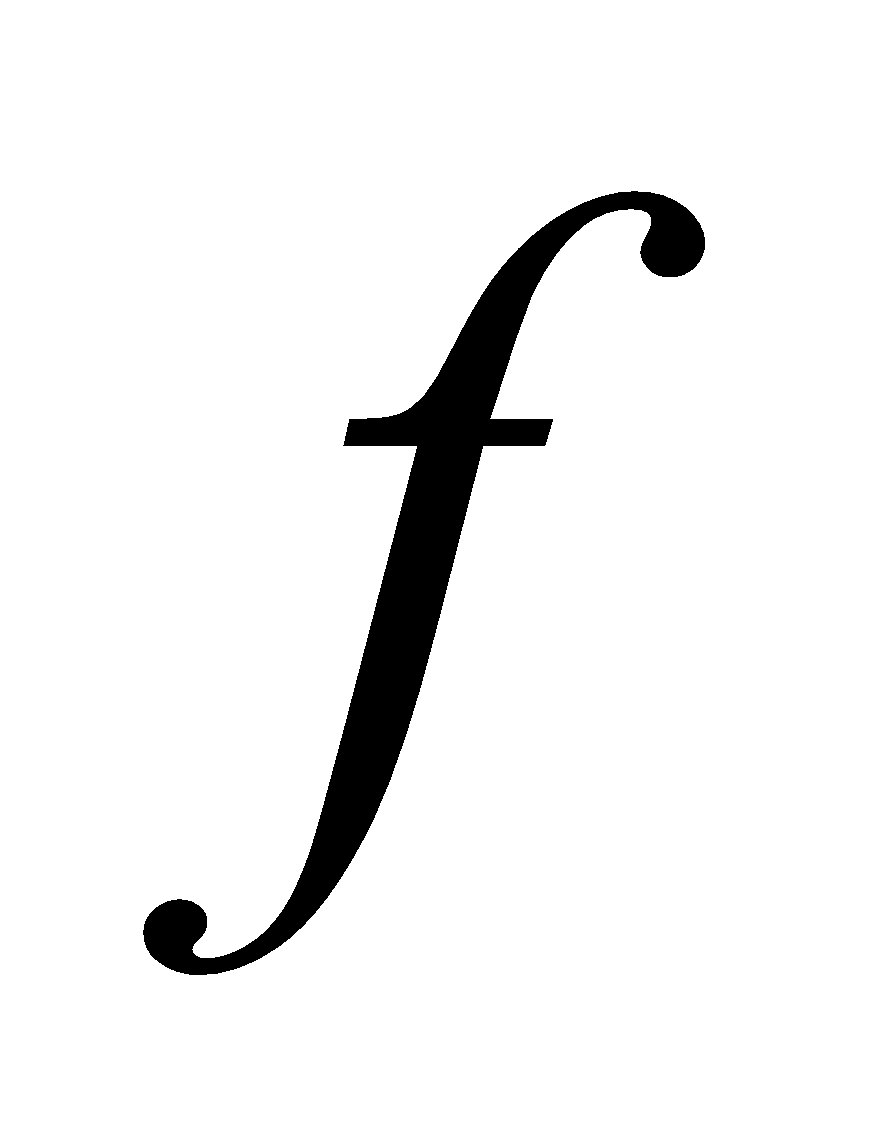and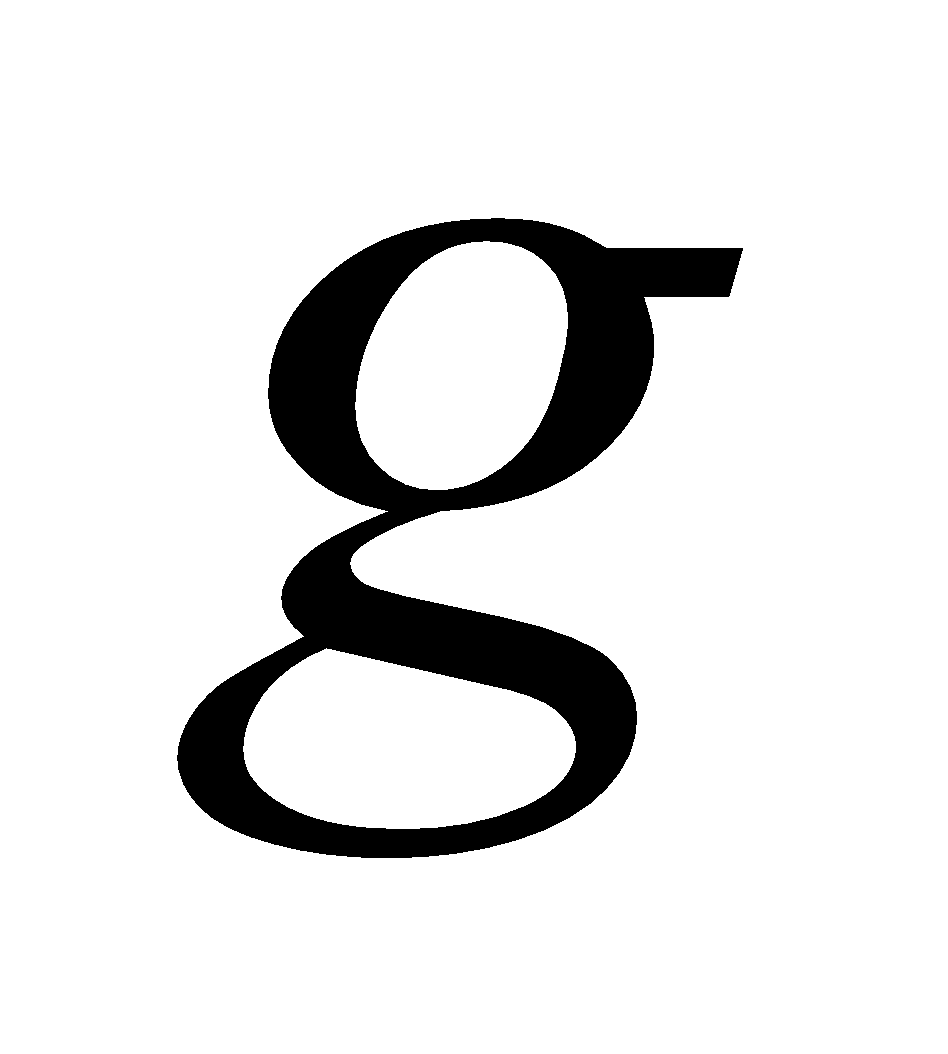are twice differentiable functions and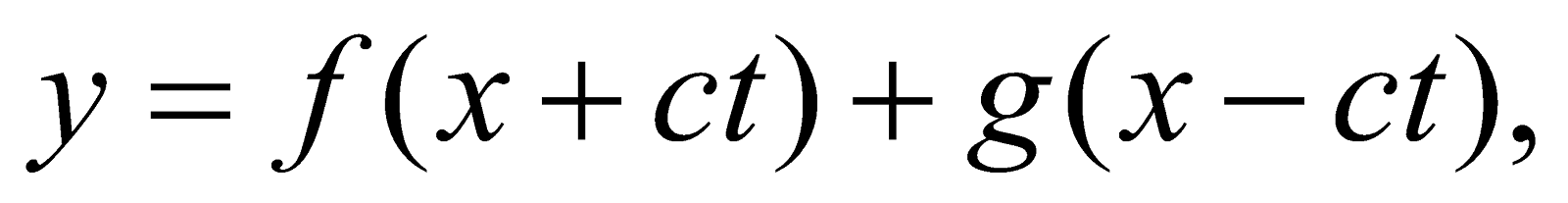then prove that 5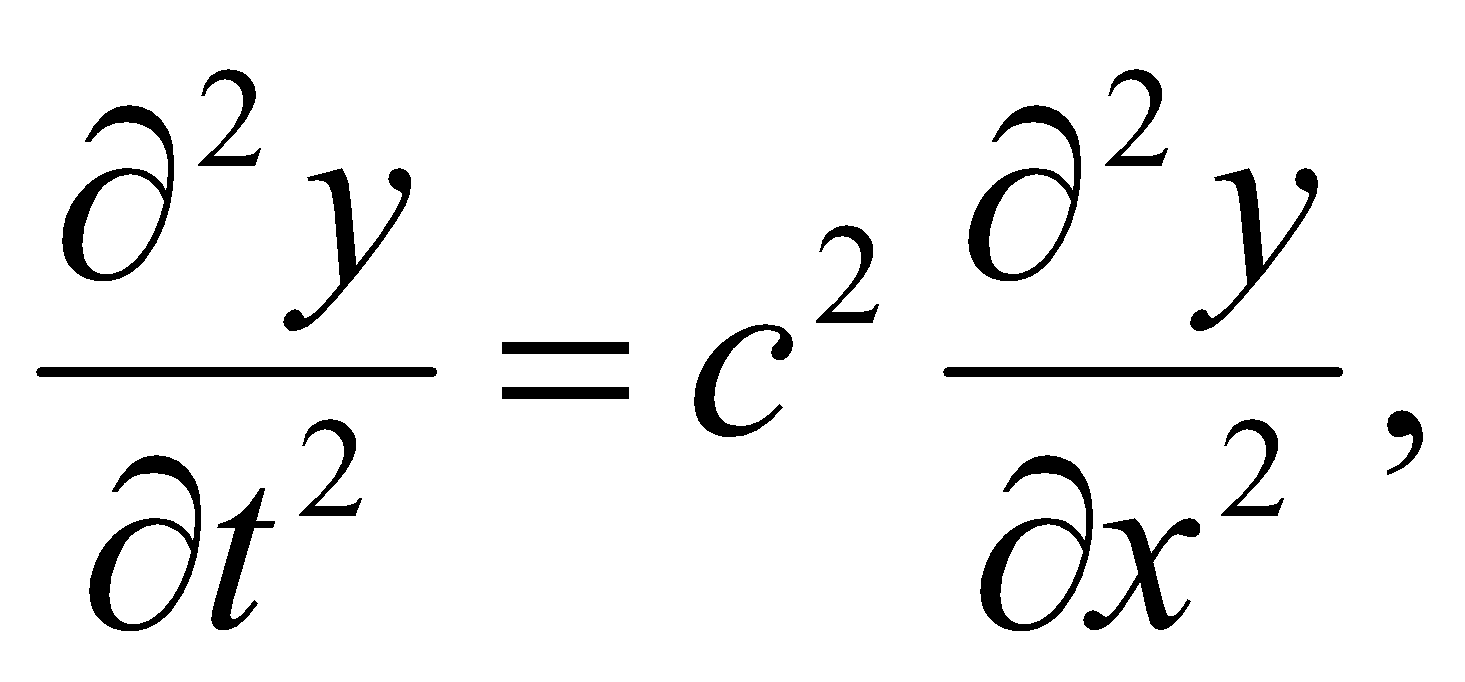c is a constant.
Or
Find the extreme values of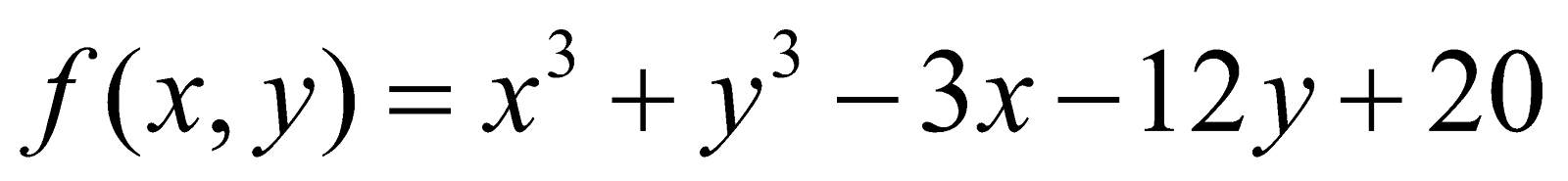GROUP – B
(Integral Calculus)
(Marks: 20)
5. (a) Write the reduction formula for 1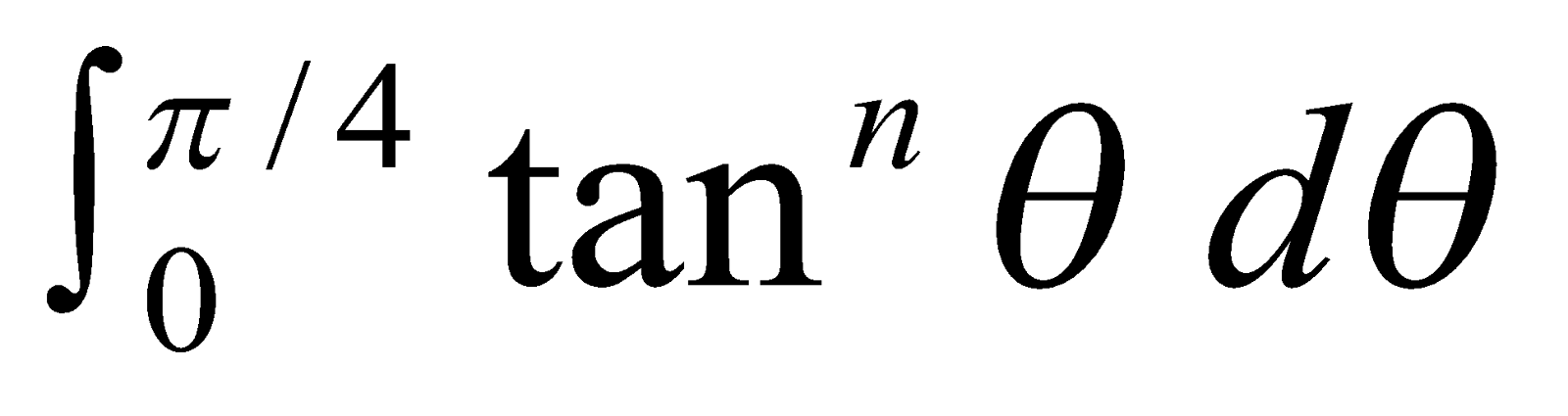(b) Prove that 2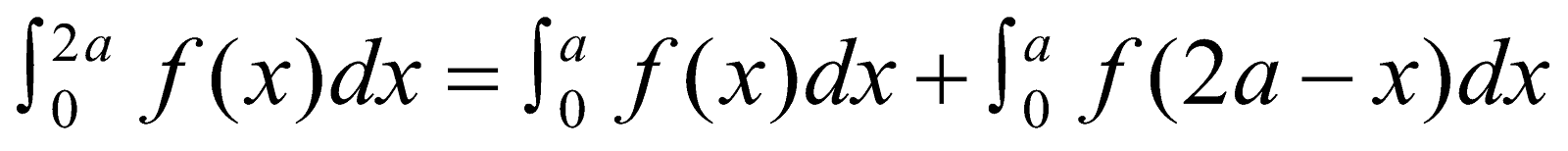(c) Show that 4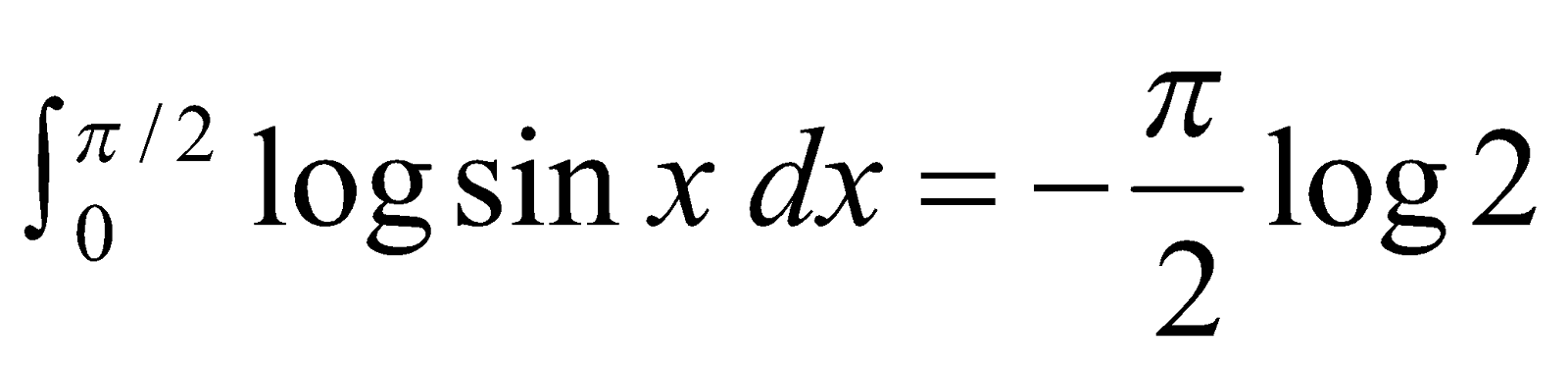(d) Evaluate: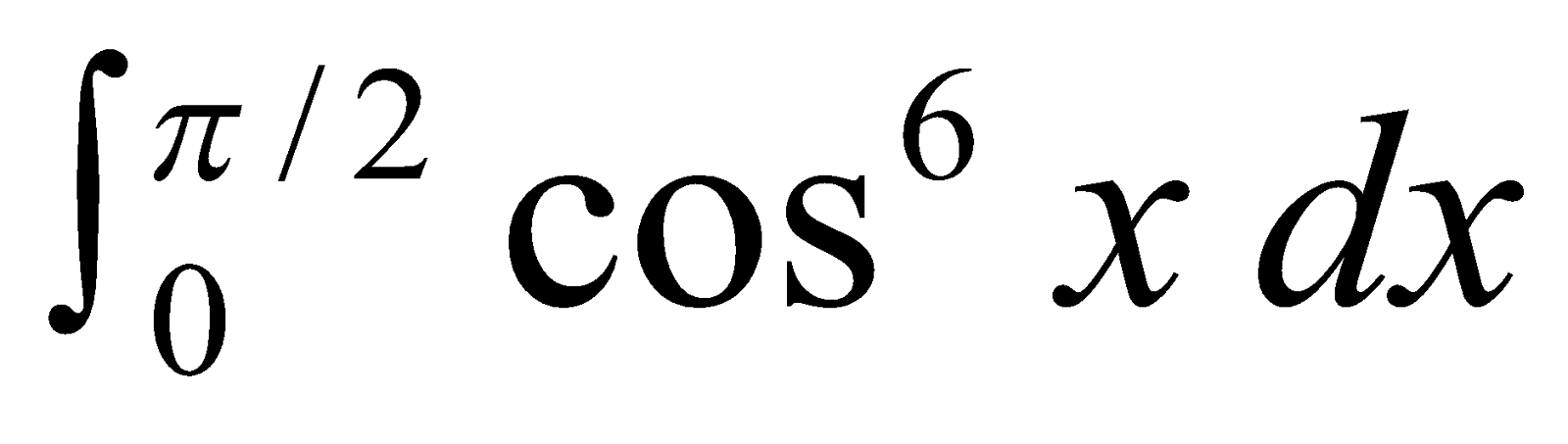Or
Prove that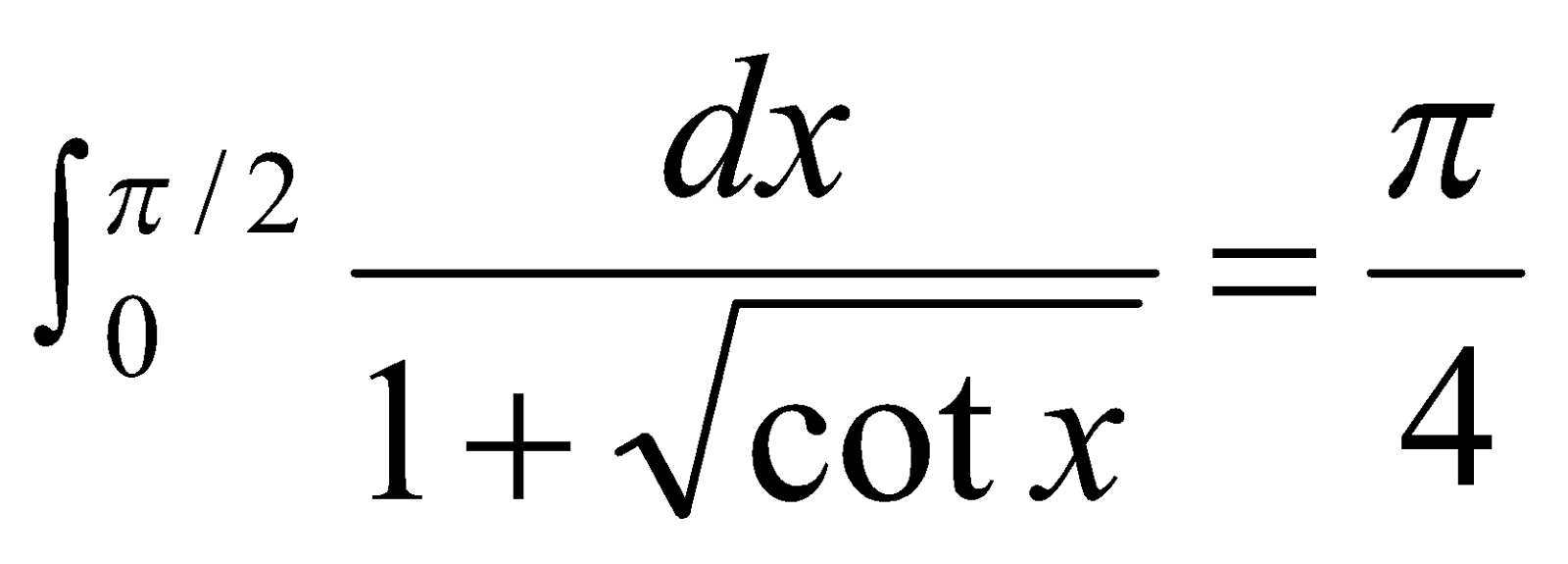6. (a) If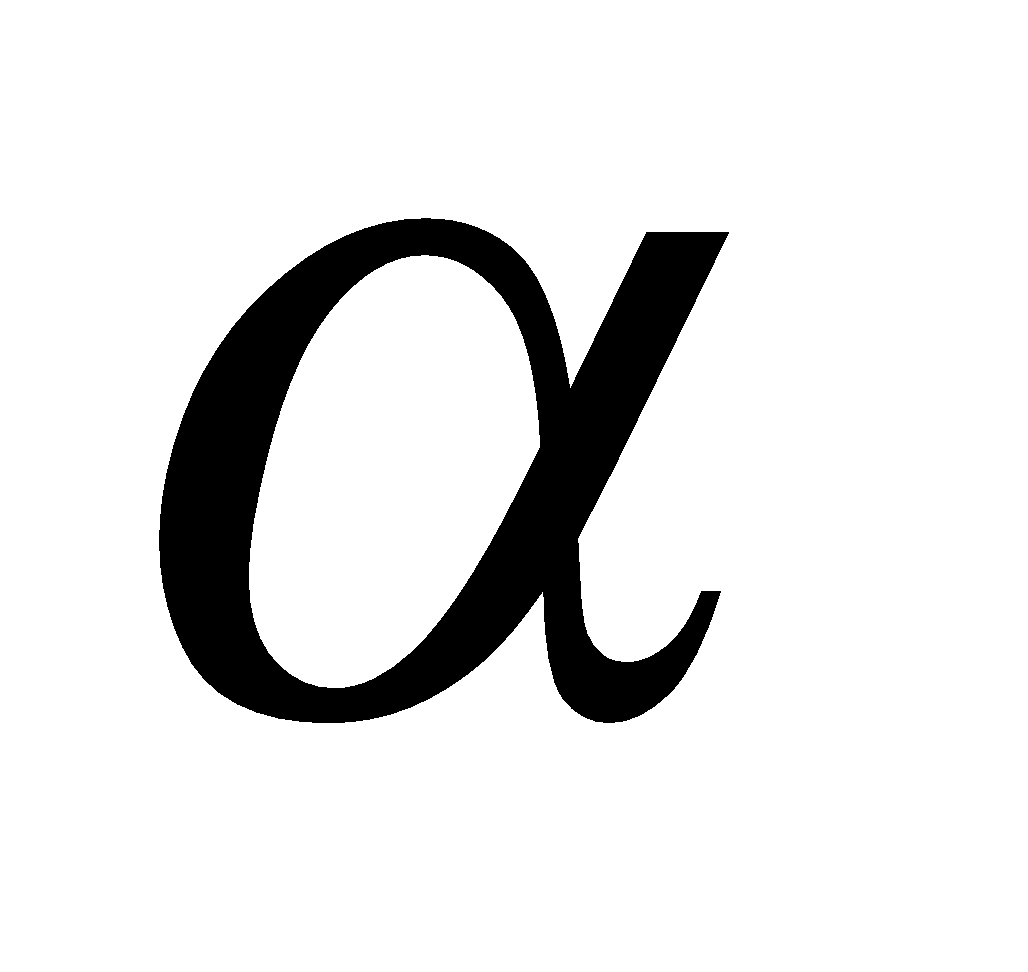and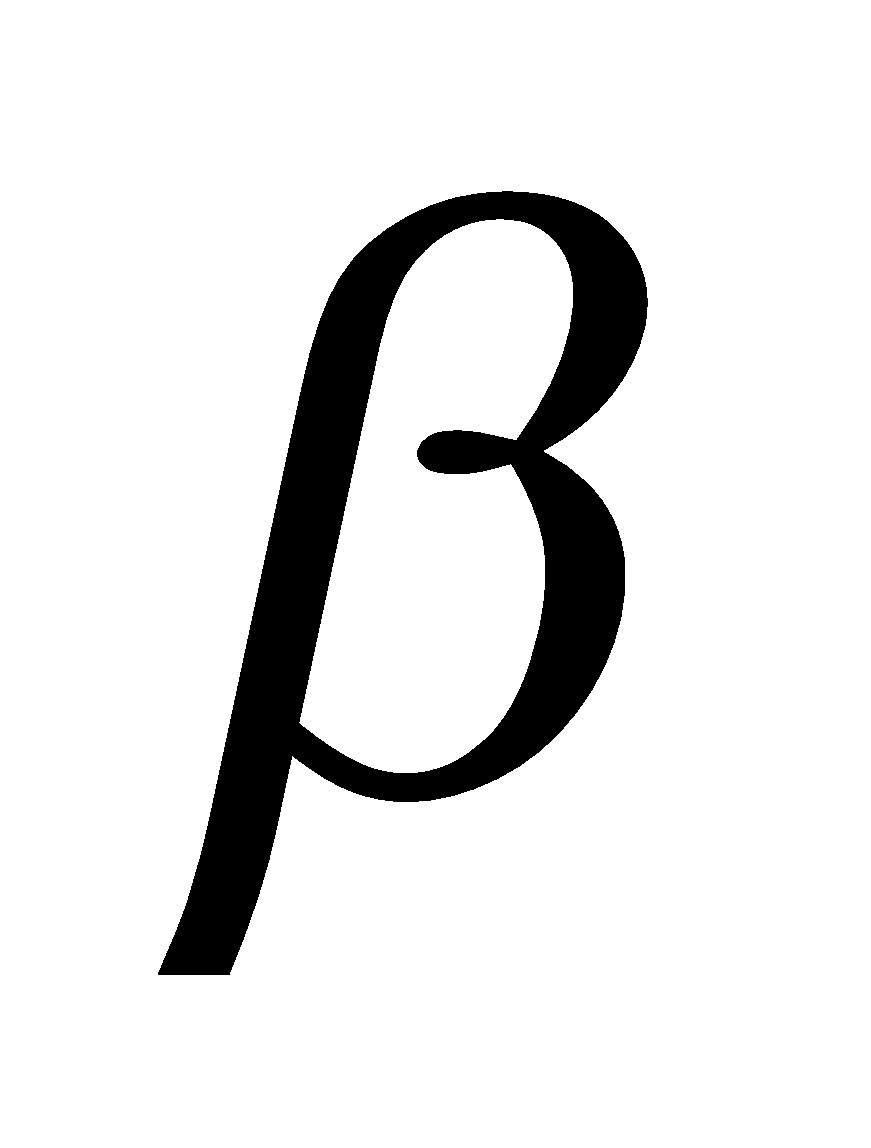be the vectorial angles of A and B respectively, then write the formula for arc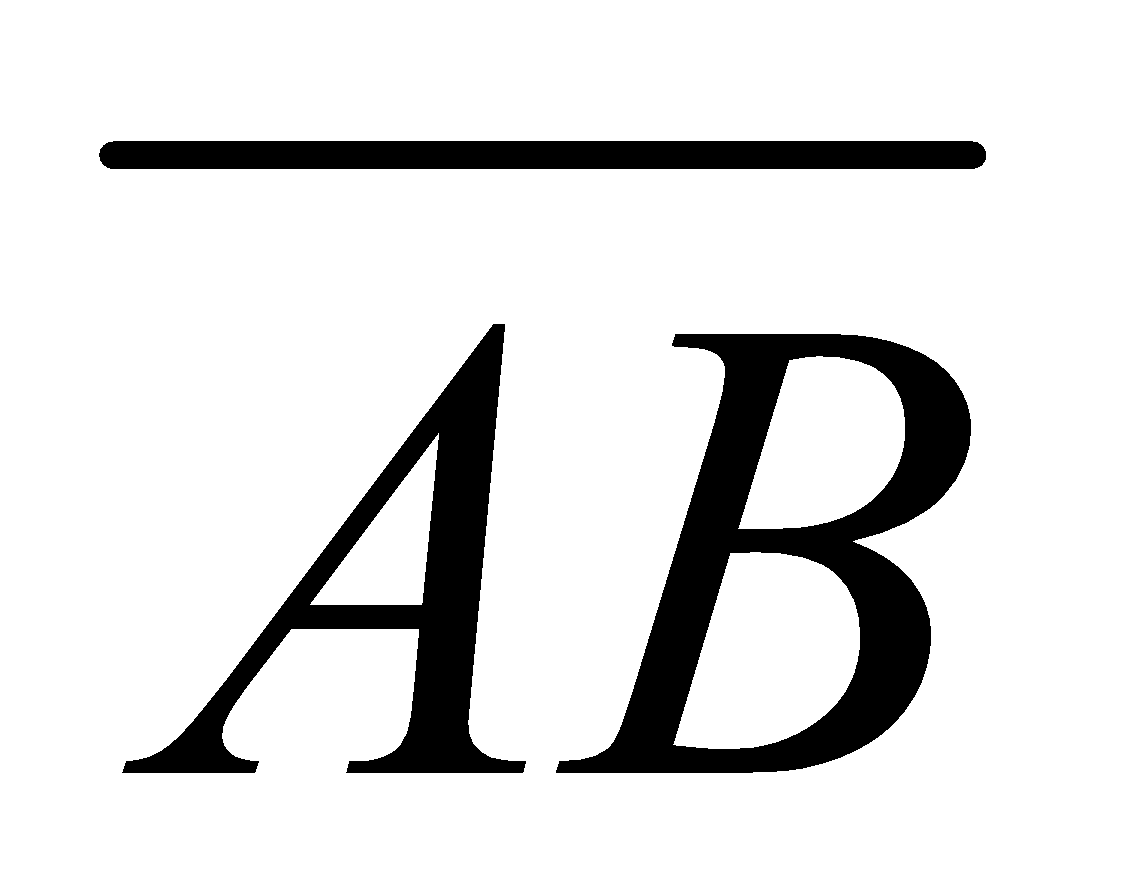1
(b) Find the perimeter of the cardioids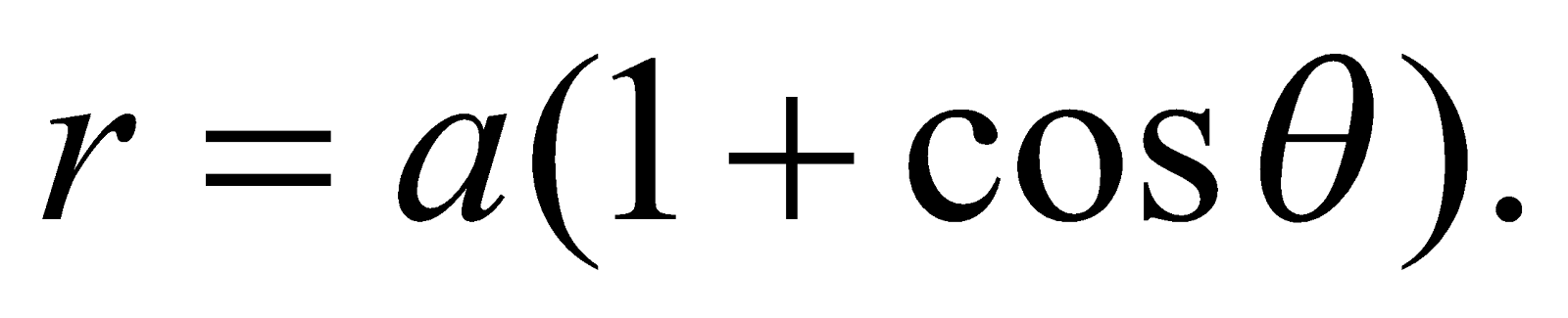5
(c) Find the area of the surface of revolution formed by revolving the curve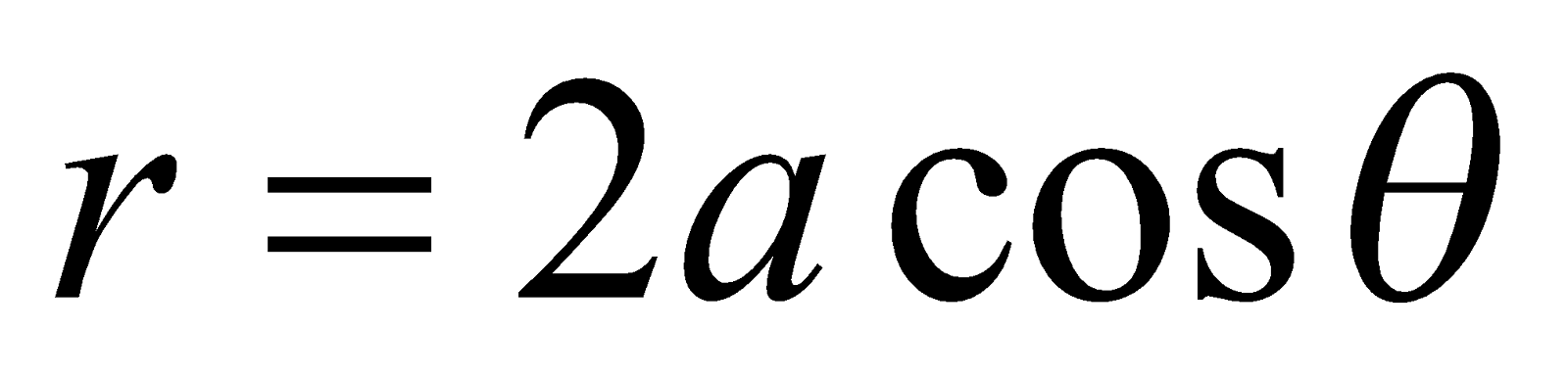about the initial line.     4
Or
Find the volume of the solid generated by revolving the asteroid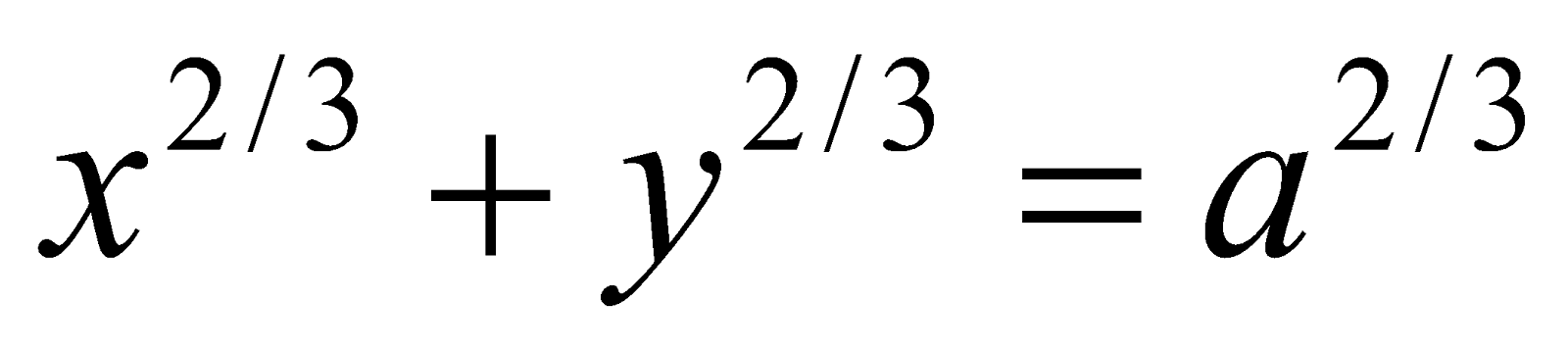GROUP – C
(Riemann Integral)
(Marks: 25)
7. (a) Define refinement of a partition. 1
(b) Show that the functiondefined by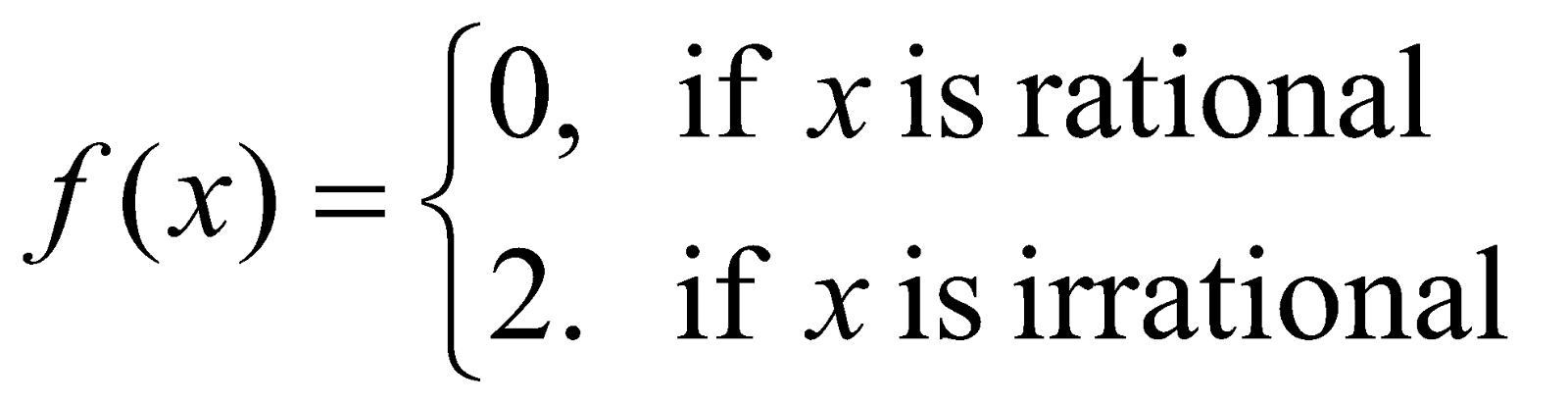is not integrable on any interval. 2
(c) Prove that every continuous function is Riemann integrable. 5
Or
If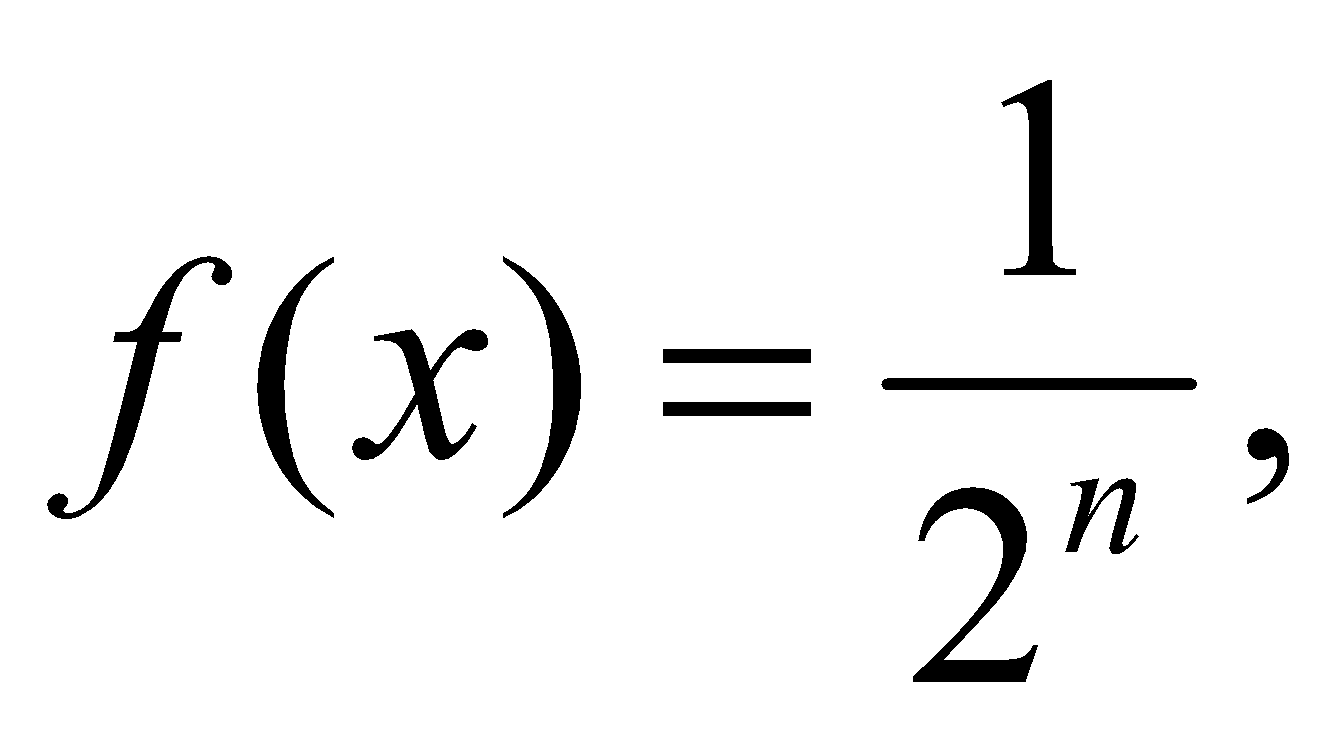when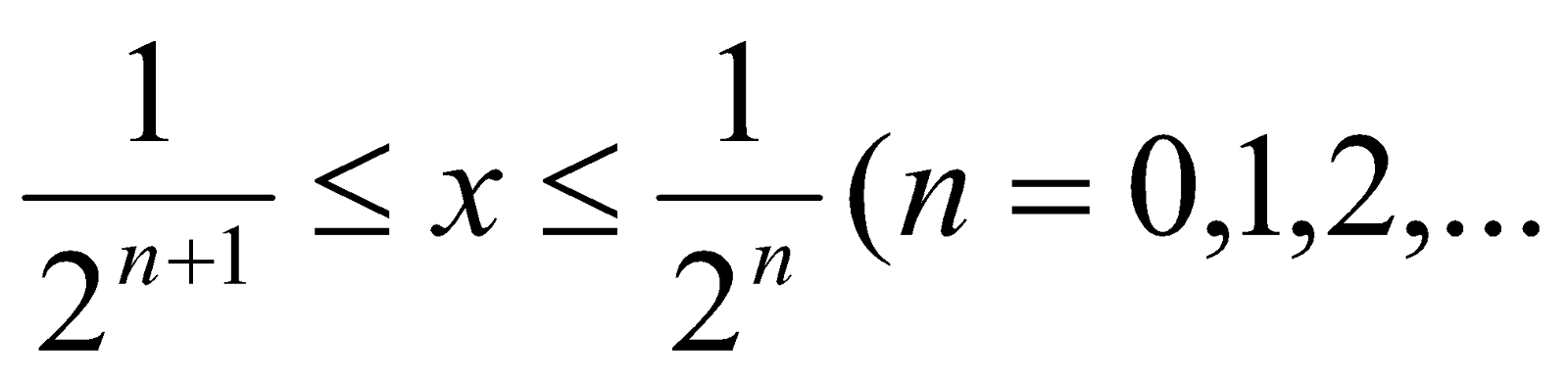)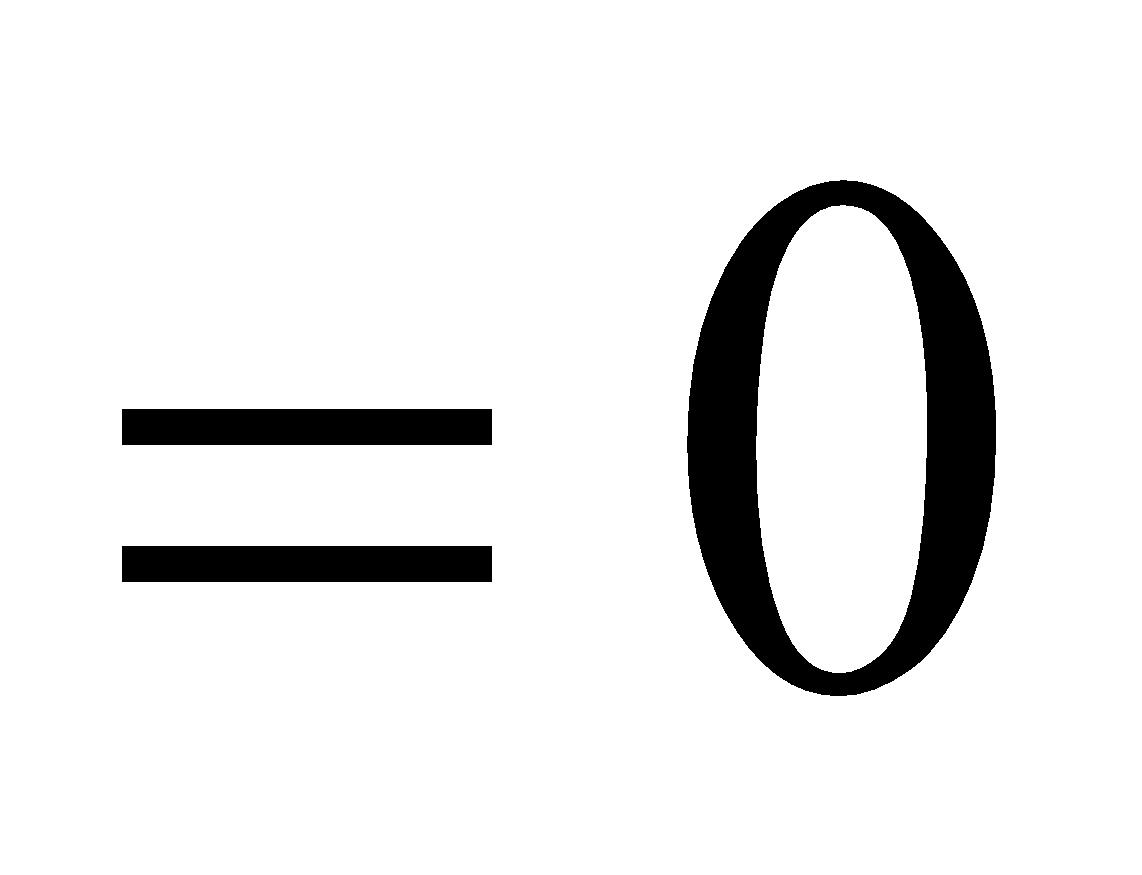, when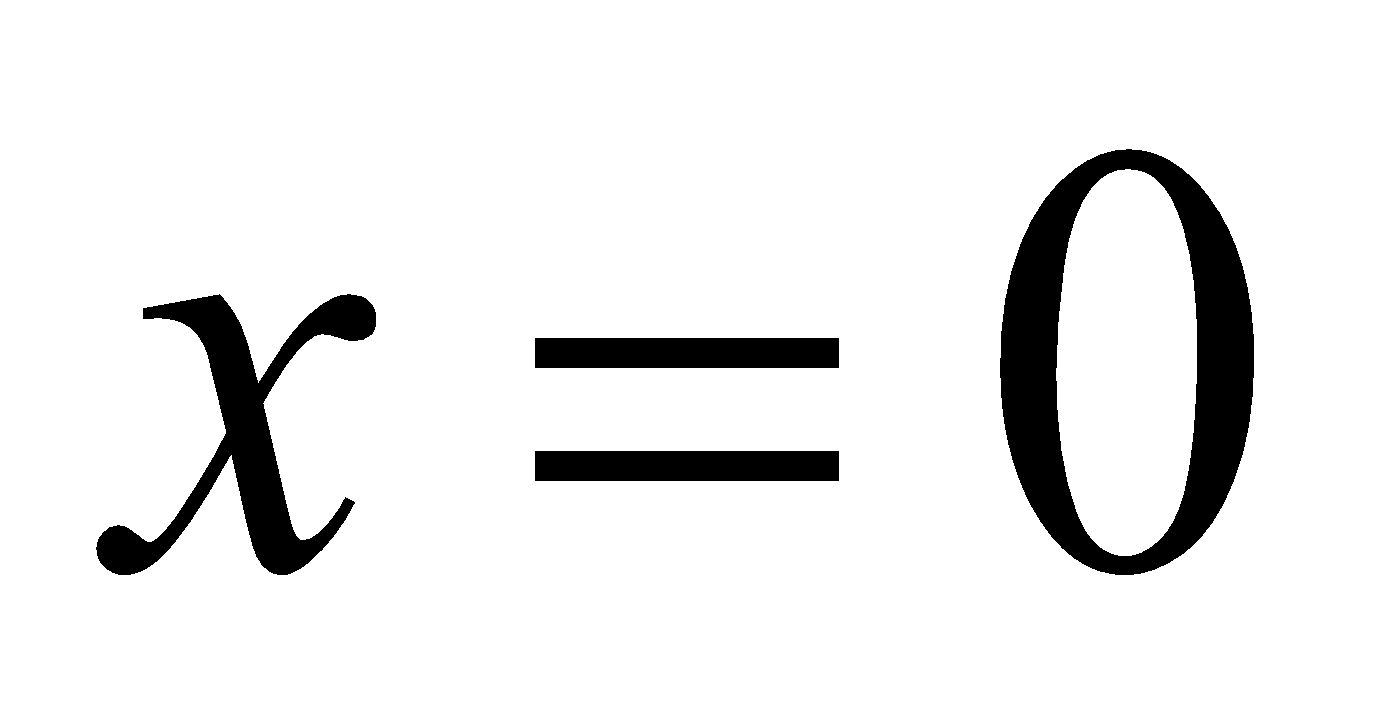then show that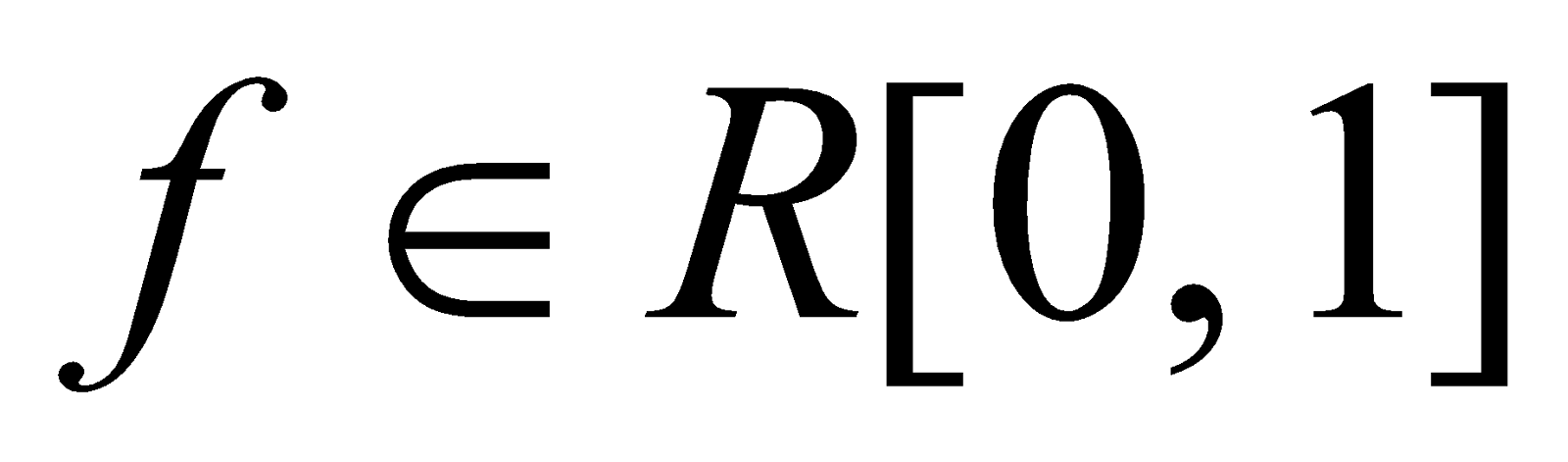although it has many points of discontinuity.

8. (a) State the fundamental theorem of integral calculus. 1
(b) Ifis a continuous function on, then show that there exists a number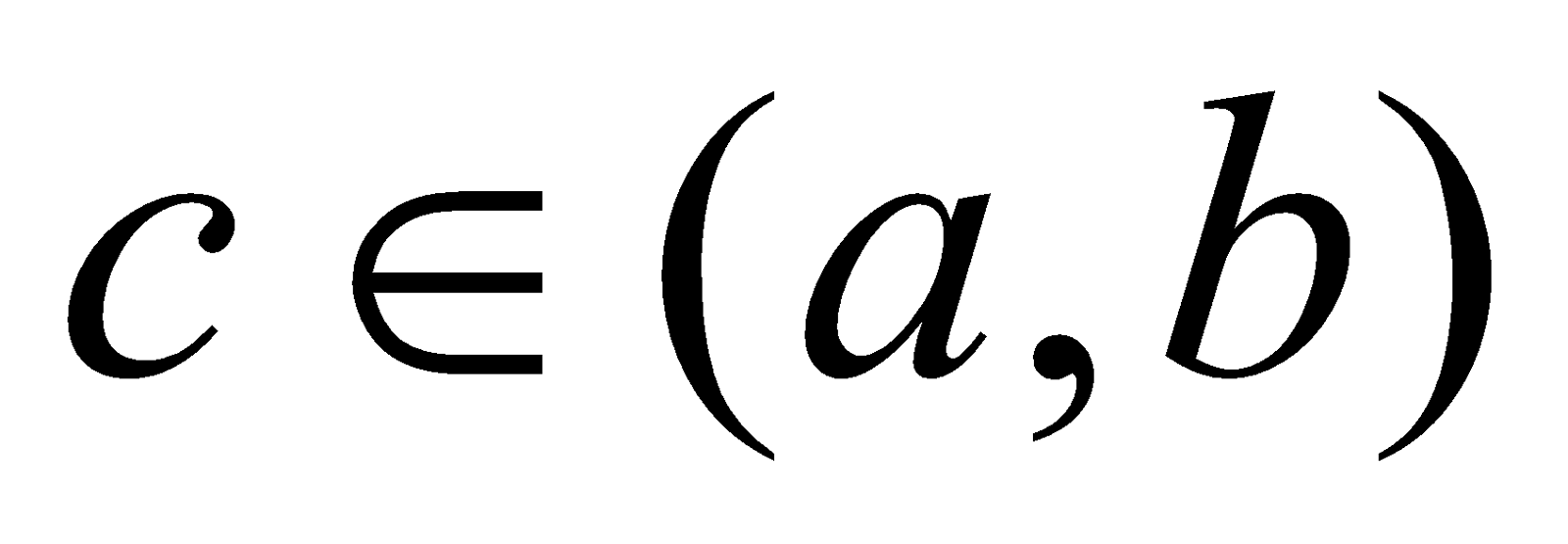such that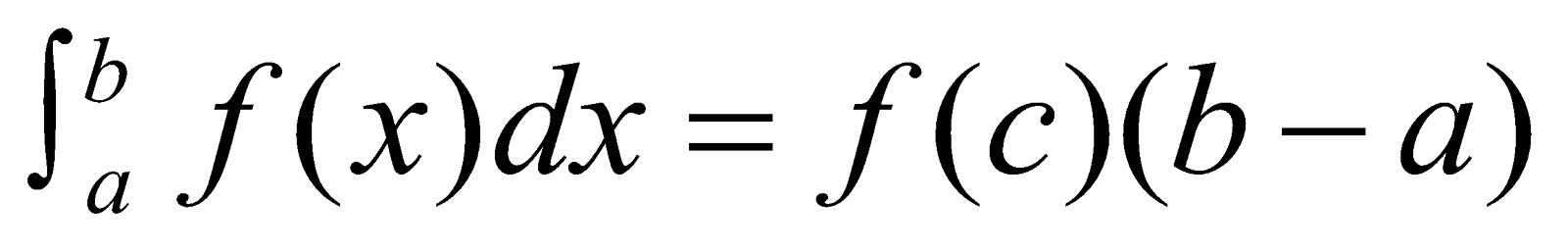3
(c) If a functionis bounded and integrable on, then the function F defined as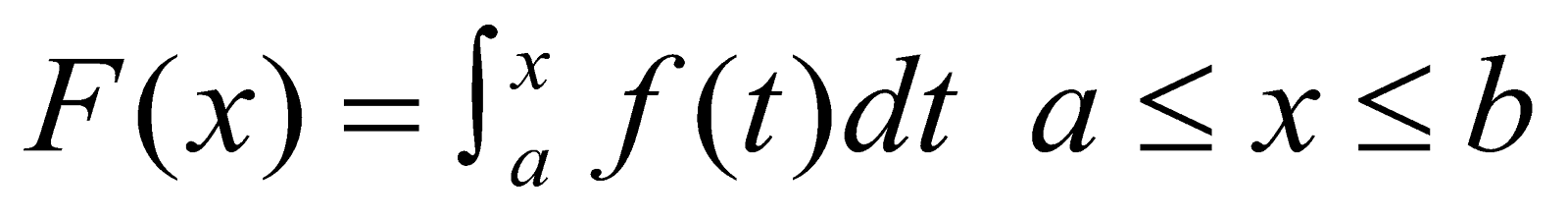Is continuous on. Prove it. 3
Or
Verify the mean value theorem for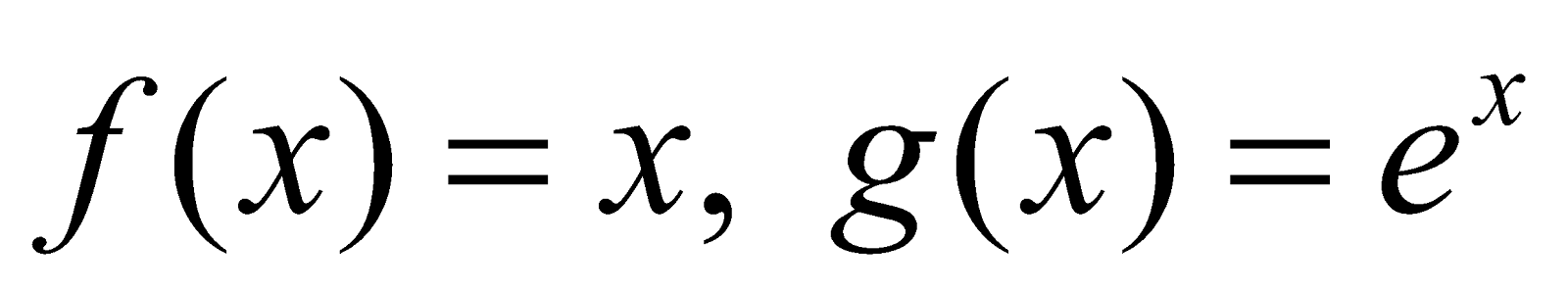in the interval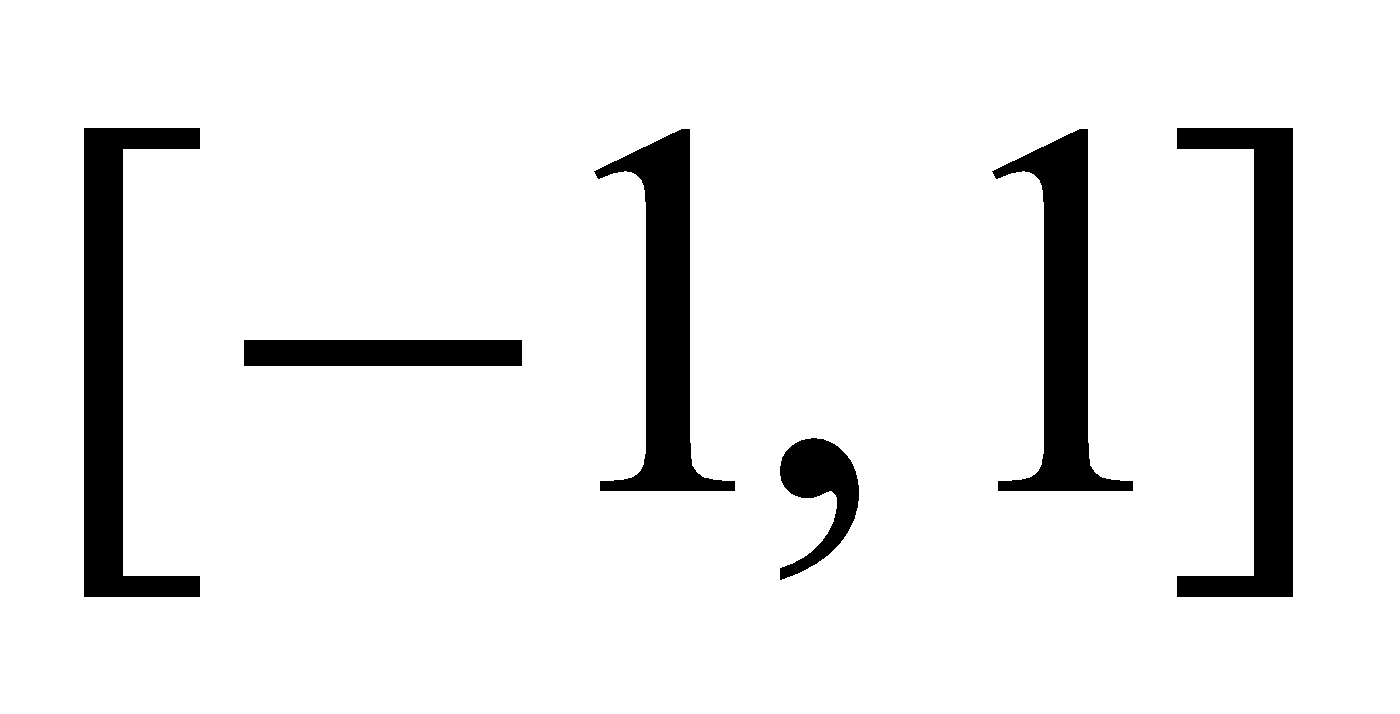.
9. (a) State the Dirichlet test for convergence of integral of a product. 1
(b) Test for convergence of 2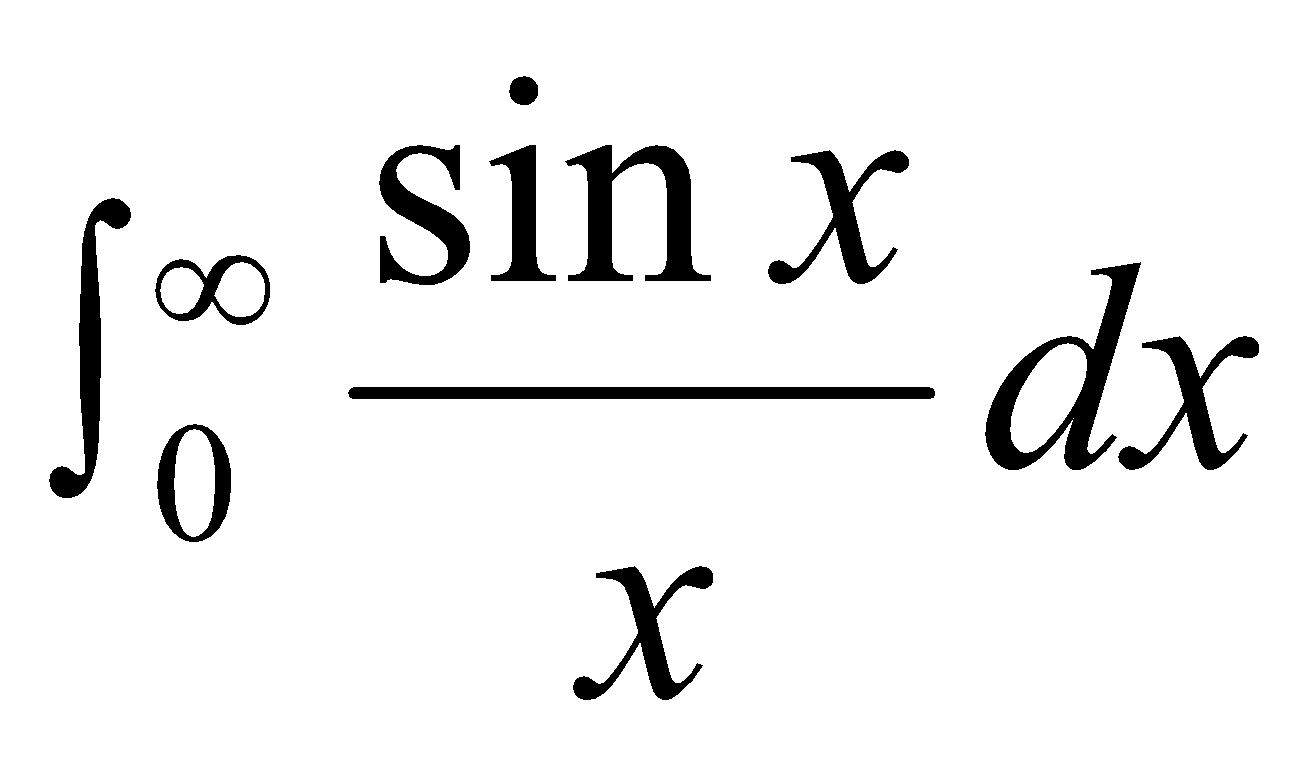(c) Prove that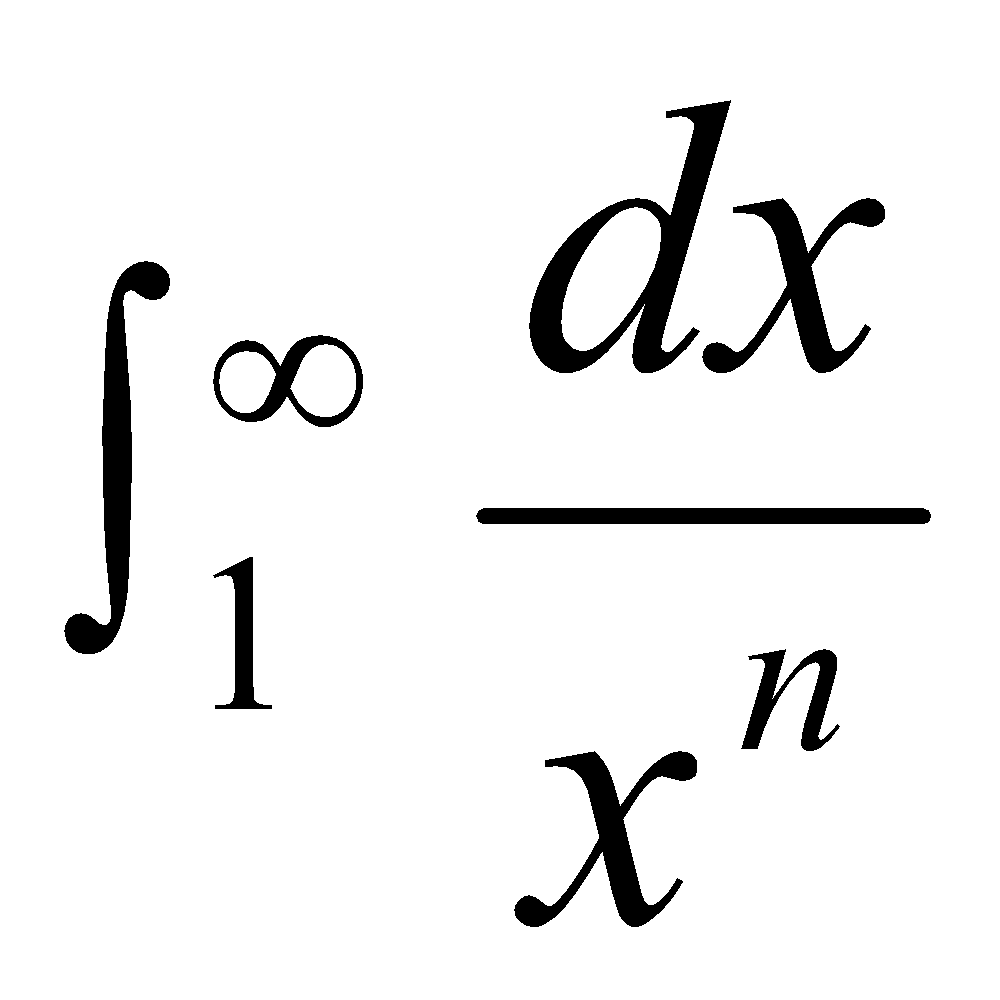is convergent for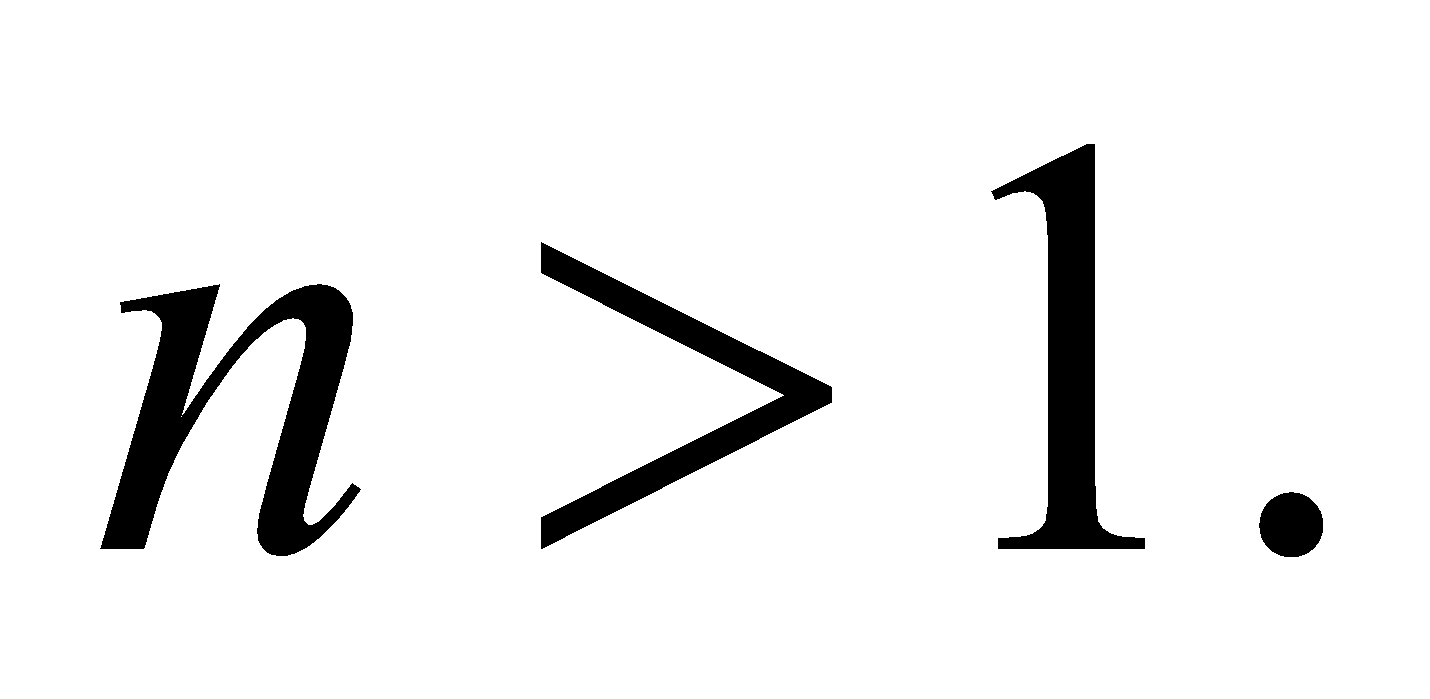3
10. Answer any one of the following: 4
(a) Prove that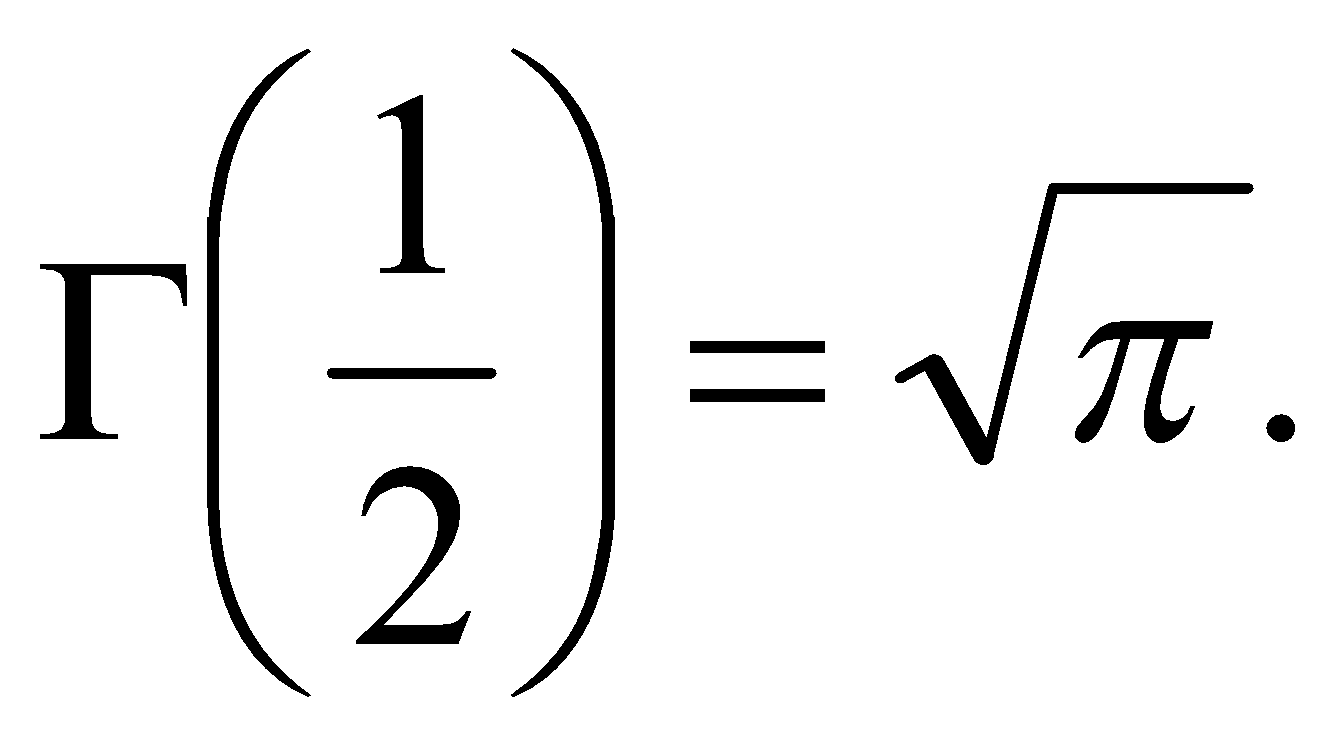(b) Prove that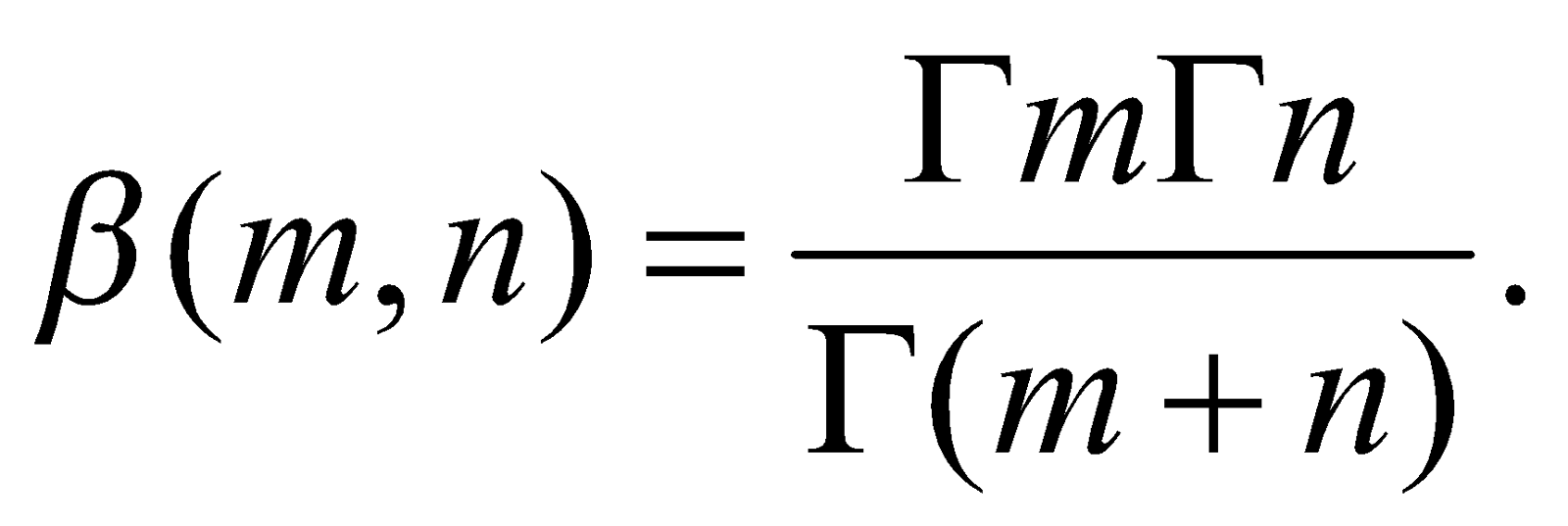***